# An introduction to wavelets using R

## 2014-01-01

### Introduction

Wavelets represent a method to decompose a signal or an image. It represents an alternative to the Fourier decomposition. It is useful in image compression, edge analysis and flexible de-noising of even complicated discontinuous functions. Although there are adaptations to more complex settings, wavelets work best when analyzing a signal that is sampled at evenly spaced time points.

To understand wavelets well, you need to refresh yourself on how the Fourier decomposition really works. Think about Fourier as a way to travel to Trigland, the land of sines and cosines.

There is data set with the wavelets package in R called “ecg” that represents 2048 evenly spaced values from a typical electrocardiogram. Here’s the code to get and graph that signal

knitr::opts_chunk$set(fig.height=1) library("ggplot2") library("magrittr") ## Warning: package 'magrittr' was built under R version 4.1.3 library("rwt") library("wavelets") data(ecg) head(ecg) ## V1 ## [1,] -0.104773 ## [2,] -0.093136 ## [3,] -0.081500 ## [4,] -0.116409 ## [5,] -0.081500 ## [6,] -0.116409 tail(ecg) ## V1 ## [1,] -0.430591 ## [2,] -0.442227 ## [3,] -0.477136 ## [4,] -0.442227 ## [5,] -0.477136 ## [6,] -0.477136 Here are some graphing functions I will need. graph_with_axis <- function(x, lab, limits=range(x)) { par(mar=c(2.6, 0, 0, 0)) plot((1:2048)/2048, x, xlim=c(0, 1.2), ylim=limits, type="l", axes=FALSE) axis(side=1, at=(0:4)/4, labels=c(1, 512, 1024, 1536, 2048)) text(1.1, x, lab, adj=0.5, cex=0.67) } graph_without_axis <- function(x, lab, limits=range(x)) { par(mar=c(0, 0, 0, 0)) plot((1:2048)/2048, x, xlim=c(0, 1.2), ylim=c(limits, limits), type="l", axes=FALSE) text(1.1, x, lab, adj=0.5, cex=0.67) } needle_plot <- function(y, lab, x=seq(0, 1, along.with=y), limits=range(y)) { par(mar=c(0, 0, 0, 0)) plot( x, y, xlim=c(0, 1.2), ylim=limits, type="n", axes=FALSE) segments(x, 0, x, y) text(1.1, 0, lab, cex=0.67) } ### The ecg signal Here’s a graph of data representing an electrocardiogram (ecg) signal that will help illustrate the use of Fourier transforms and wavelets. graph_with_axis(ecg, "ecg\nsignal")Figure 1. Graph of ecg signal You can ignore the units of the signal itself, as they are arbitrary (at least for this application), but the minimum value is -1.48 and the maximum is 1.44. ### Fourier transforms You compute the Fourier decomposition using the fft command, which is part of the base R package. The letters stand for “fast fourier transform”. ft.ecg <- fft(ecg)/2048 head(ft.ecg) ## V1 ## [1,] -0.040859772+0.000000000i ## [2,] -0.144005054-0.128781054i ## [3,] -0.004311727+0.007706751i ## [4,] 0.023643179+0.046245122i ## [5,] 0.004964307-0.026597976i ## [6,] -0.010241779+0.017355456i tail(ft.ecg) ## V1 ## [1,] 0.010344229+0.000402821i ## [2,] -0.010241779-0.017355456i ## [3,] 0.004964307+0.026597976i ## [4,] 0.023643179-0.046245122i ## [5,] -0.004311727-0.007706751i ## [6,] -0.144005054+0.128781054i This function produces complex numbers, which have an interpretation in terms of sine and cosine functions. par(mar=c(0,0,0,0)) plot(Re(ft.ecg), Im(ft.ecg), axes=FALSE, type="n") text(Re(ft.ecg), Im(ft.ecg), 1:2048)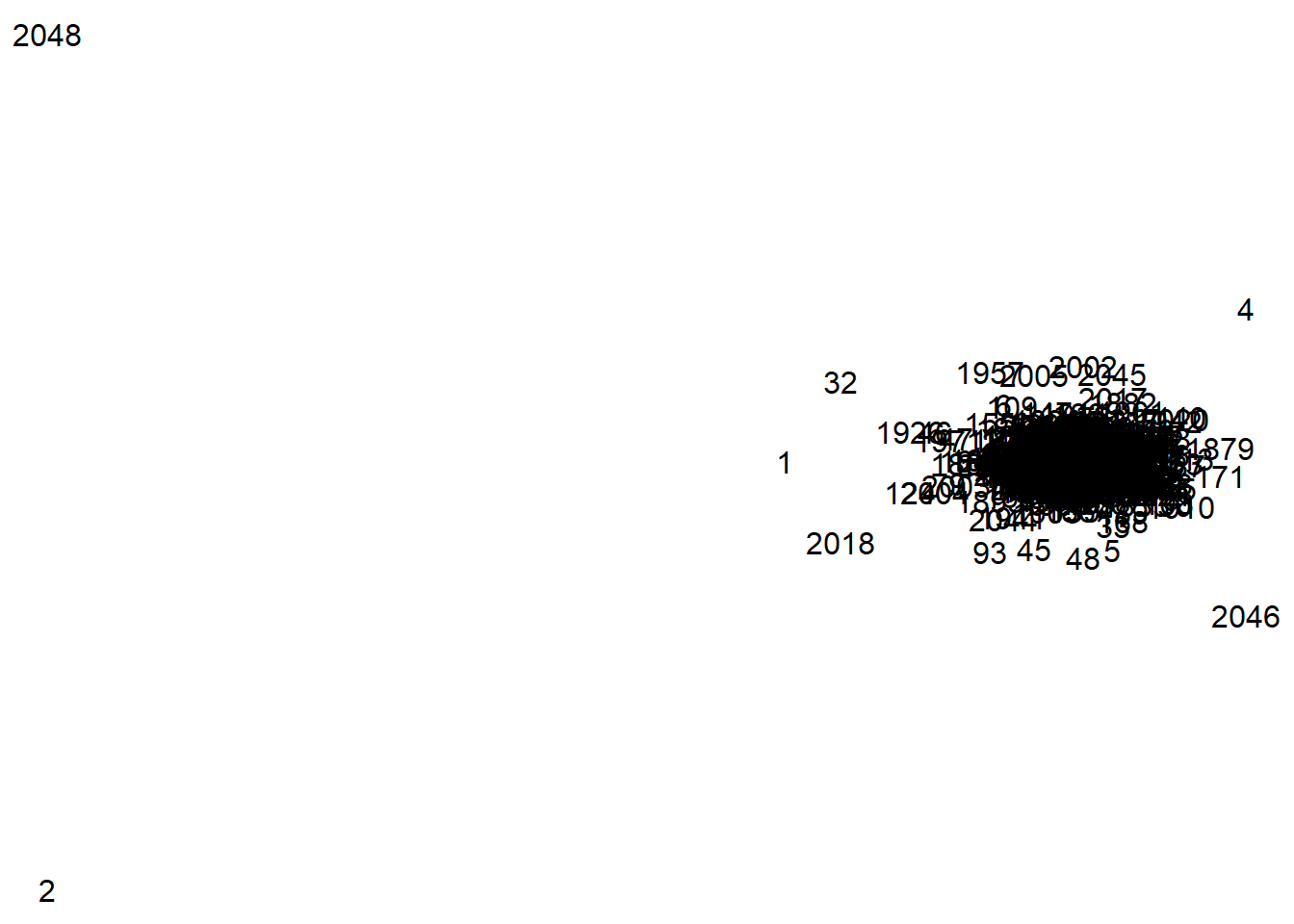Here is a plot of the coefficients on the complex plane. Notice that certain coefficients (1, 2, 4, 32, 2018, and 2046) are further from the origin. ### Inverse Fourier transform ift.ecg <- fft(ft.ecg,inverse=TRUE) head(ift.ecg) ## V1 ## [1,] -0.104773-0i ## [2,] -0.093136+0i ## [3,] -0.081500-0i ## [4,] -0.116409+0i ## [5,] -0.081500-0i ## [6,] -0.116409+0i tail(ift.ecg) ## V1 ## [1,] -0.430591+0i ## [2,] -0.442227+0i ## [3,] -0.477136+0i ## [4,] -0.442227-0i ## [5,] -0.477136-0i ## [6,] -0.477136-0i You convert from the Fourier coefficients back to the original signal using the same fft function, but this time with an inverse=TRUE argument. ### Components of the Fourier transform The object ft.ecg contains the Fourier decomposition, and ift.ecg is the inverse of the decomposition. You need to scale the Fourier decomposition by the number of data points (2,048). The inverse gets the original signal (-0.104773-0i, , -0.0931359999999999+0i, , -0.0815-0i, , …) except that there is a “+0i” or “-0i” tacked on to the end. The complex numbers in the Fourier decomposition are a short-hand for the mix of sines and cosines. The first number has only a real component and no imaginary component. This component represent the mean of the entire signal. mean(ecg) ##  -0.04085977 ft.ecg ##  -0.04085977+0i For the remaining numbers, the real part represents the cosine function and the imaginary part represents the sine function. So -0.144005053923261-0.128781053603887i represents -0.1440051 cos(2pit) and -0.1287811 sin(2pit). The following code shows the graph of the cosine function, the sine function and the sum. t.unit <- (1:2048)/2048 cos1 <- +Re(ft.ecg)*cos(2*pi*t.unit) sin1 <- -Im(ft.ecg)*sin(2*pi*t.unit) sum1 <- cos1+sin1 { graph_without_axis(cos1, "Cosine\ncomponent", limits=range(sum1)) graph_without_axis(sin1, "Sine\ncomponent", limits=range(sum1)) graph_without_axis(sum1, "Combined\ncomponent", limits=range(sum1)) }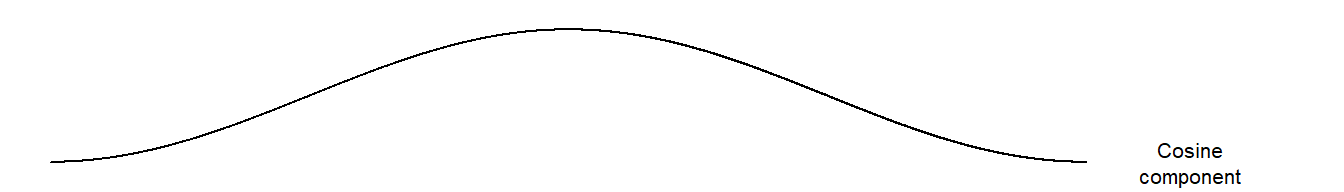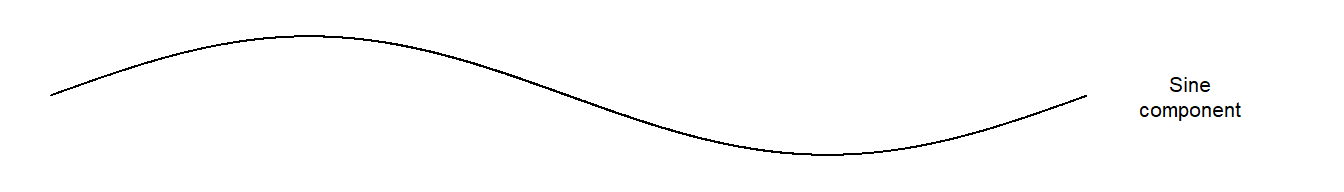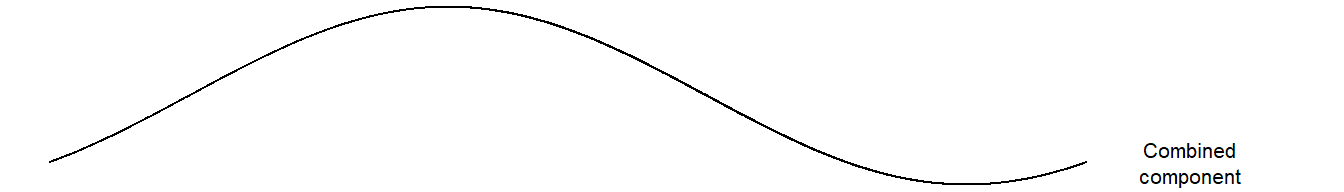The cosine function is a wave with a peak in the middle and a trough at the beginning (or equivalently, the end). This is actually the reverse of a cosine function, but only because the coefficient is negative. The sine function is a wave with a peak a quarter of the way through and trough three quarters of the way through. A weighted sum of sine and cosine fuctions produces a trough at a different location. Here is about a third of the way through. If you look at the original signal, the peak and trough roughly match the shape of the ecg curve, if you ignore the sudden spikes. { graph_without_axis(sum1, "Combined\ncomponent") graph_without_axis(ecg, "Original\nsignal") }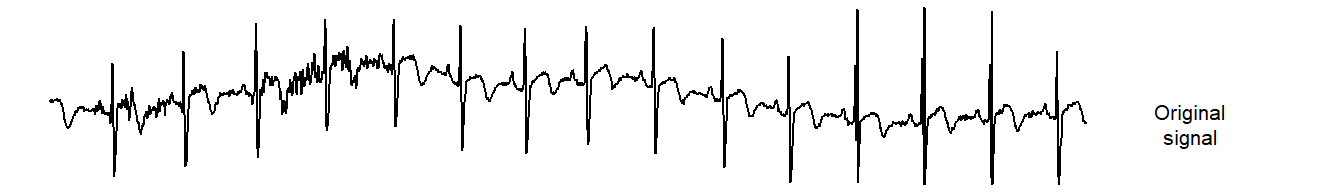The sum shows the peak for the sum that is somewhere between the peaks of the sine and cosine functions. It’s actually slightly closer to the peak of the cosine function, but not by much. The trough is also between the two troughs. The relative weights given to the sine and cosine functions and the positive or negative values associated with these weights will control where the peak and trough occur for the sum. In other words, you can control the phase of the curve. If you are familiar with the Mod and Arg functions, they can help you compute the phase (the location of the peak/trough) and the amplitude (the height at the peak). Now this was a lot of work to decode the second component of the Fourier decomposition. You might forget to scale by the length of the signal. You could reverse the sines and cosines. Yu might compute the sines and cosines on a range from -pi to pi instead of from 0 to 2pi. You might leave out an important minus sign. Trust me, I did all these things. ### Zeroing out components There’s a faster and more convenient way. Let the computer do the work for you. Take the Fourier decomposition, zero out everything except the second component, and then invert the decomposition. This allows you to graph the second component directly. ft.comp2 <- ft.ecg ft.comp2[-2] <- 0 comp2 <- Re(fft(ft.comp2,inverse=TRUE)) graph_without_axis(sum1, "Using\nInverse fft") { lines((1:2048)/2048, sum1, col="red", lty="dashed", lwd=3) }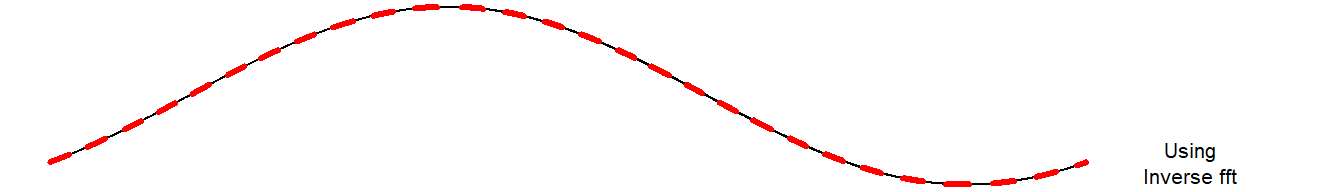This is the graph. You can use the same method to show the shape of the third Fourier component. The third component has sines and cosines, but the period is two. -0.0043117 cos(2pit/2) and 0.0077068 sin(2pit/2). ft.comp3 <- ft.ecg ft.comp3[-3] <- 0 comp3 <- Re(fft(ft.comp3,inverse=TRUE)) graph_without_axis(comp3, "Third\ncomponent")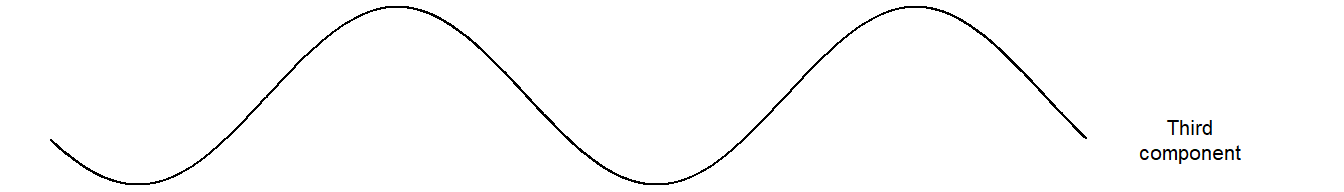This component has a higher frequency, with two peaks rather than one. Do the same for the fourth Fourier component ft.comp4 <- ft.ecg ft.comp4[-4] <- 0 comp4 <- Re(fft(ft.comp4,inverse=TRUE)) graph_without_axis(comp4, "Fourth\ncomponent")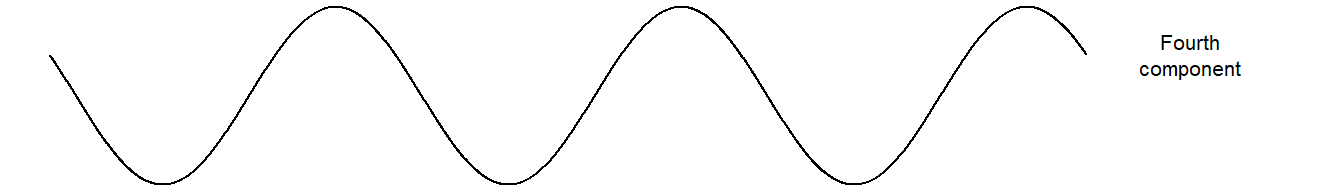The fourth Fourier component has three peaks. ### Orthogonality The interesting thing is that the second and third and fourth Fourier components are orthogonal to one another. Actually, every Fourier component is orthogonal to every other Fourier component. Mathematical folks like “orthogonal” because it allows you to derive the mathematical properties of the decomposition with greater ease. For a practical person, what “orthogonal” means is that the information contained in one Fourier component does not “overlap” with that in any other Fourier component. It also means you can run the decomposition in any order (low frequency to high frequency, high frequency to low frequency, or even a haphazard frequency). Finally, orthogonality also preserves independence properties in certain settings. The Fourier decomposition continues with components of higher and higher frequency. The following code computes the 32nd component of the Fourier transform. ft.comp32 <- ft.ecg ft.comp32[-32] <- 0 comp32 <- Re(fft(ft.comp32,inverse=TRUE)) graph_without_axis(comp32, "32nd\ncomponent")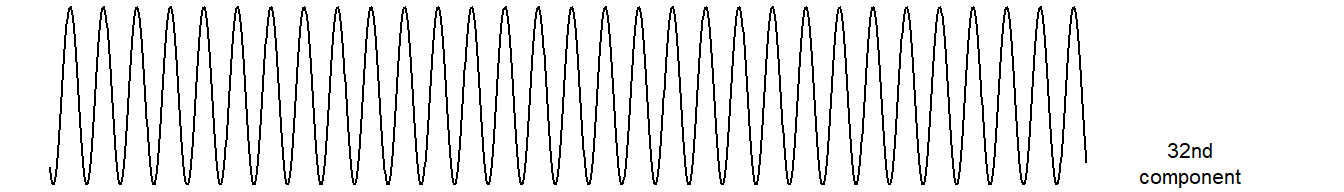Here’s the 32nd Fourier component, and if you count carefully, you will get 31 peaks. Count them carefully. No, don’t bother, I’ll do it for you. Because that are packed so closely together, I will only show you every third peak. m <- max(comp32) graph_without_axis(comp32, "32nd\ncomponent", limits=c(-m, 1.2*m)) text((seq(1, 31, by=3)-0.5)/31, 1.2*m, seq(1, 31, by=3), col="red", cex=0.67)If you continue down the list, you get a higher and higher frequency or equivalently more and more peaks. ### Wavelets Now wavelets produce a deocomposition much like the Fourier decomposition but wavelets are different in one important way. Wavelets break the signal up into pieces and model signals within those pieces. The frequency of the wavelet signal is associated with the number of pieces. For high frequency wavelets, you break the signal up into a large number of pieces. For low frequency signals, you breah the signal up into fewer pieces. There are at least two good R packages for wavelet docompositon, rwt and wavelets. Here’s a brief overview of how rwt works. Perhaps the most famous of the wavelets is the Daubechies 4 wavelet. d4.rwt <- daubcqf(4) d4.rwt ##$h.0
##   0.4829629  0.8365163  0.2241439 -0.1294095
##
## $h.1 ##  0.1294095 0.2241439 -0.8365163 0.4829629 wt.rwt <- mdwt(ecg,daubcqf(4)$h.0,L=9)
names(wt.rwt)
##  "y" "L"
head(wt.rwt$y) ## [,1] ## [1,] 0.797545 ## [2,] 6.979219 ## [3,] -2.933776 ## [4,] -8.541192 ## [5,] 4.087582 ## [6,] 2.536293 tail(wt.rwt$y)
##                 [,1]
## [2043,]  0.003011394
## [2044,] -0.012342195
## [2045,]  0.001102199
## [2046,] -0.030707757
## [2047,] -0.007824638
## [2048,]  0.126029962
wt.rwt$L ##  9 iwt.rwt <- midwt(wt.rwt$y,daubcqf(4)$h.0,L=9) names(iwt.rwt) ##  "x" "L" head(iwt.rwt$x)
##           [,1]
## [1,] -0.104773
## [2,] -0.093136
## [3,] -0.081500
## [4,] -0.116409
## [5,] -0.081500
## [6,] -0.116409
tail(iwt.rwt$x) ## [,1] ## [2043,] -0.430591 ## [2044,] -0.442227 ## [2045,] -0.477136 ## [2046,] -0.442227 ## [2047,] -0.477136 ## [2048,] -0.477136 iwt.rwt$L
##  9

With the rwt package, you choose the particular wavelet decomposition using the daubcqf function. The wavelet decomposition uses the mdwt function and the inverse of the wavelet decomposition uses the midwt decomposition. The rwt package is fairly minimal with a family of Duabechies wavelets, but no alternatives (such as coiflets). Don’t ask me what a coiflet is.

### wavelets package

The wavelets package is more comprehensive, but also more complex.

d4.wavelets <- wt.filter("d4")
d4.wavelets
## An object of class "wt.filter"
## Slot "L":
##  4
##
## Slot "level":
##  1
##
## Slot "h":
##  -0.1294095 -0.2241439  0.8365163 -0.4829629
##
## Slot "g":
##   0.4829629  0.8365163  0.2241439 -0.1294095
##
## Slot "wt.class":
##  "Daubechies"
##
## Slot "wt.name":
##  "d4"
##
## Slot "transform":
##  "dwt"
wt.wavelets <- dwt(ecg,filter="d4")
slotNames(wt.wavelets)
##   "W"          "V"          "filter"     "level"      "n.boundary"
##   "boundary"   "series"     "class.X"    "attr.X"     "aligned"
##  "coe"
iwt.wavelets <- idwt(wt.wavelets)
head(iwt.wavelets)
##            V1
## [1,] -0.10477
## [2,] -0.09314
## [3,] -0.08150
## [4,] -0.11641
## [5,] -0.08150
## [6,] -0.11641
tail(iwt.wavelets)
##            V1
## [1,] -0.43059
## [2,] -0.44223
## [3,] -0.47714
## [4,] -0.44223
## [5,] -0.47714
## [6,] -0.47714

With the wavelets package, you choose the particular wavelet decomposition using the wt.filter function. The wavelet decomposition uses the dwt function and the inverse of the wavelet decomposition uses the idwt function. Notice that the wavelets package uses S4 objects.

I will use the rwt package for the rest of my examples. The S4 objects in wavelets are difficult to manipulate directly, and I could not get some of the hand calculations to match what this package computed. I’m sure the failure to get a match with my hand calculations is just because of my limitations.

I will also use the d4 or Daubechies 4 wavelet for these examples. Ingird Daubechies is one of the pioneers in wavelet theory and she developed a series of wavelets, d2, d4, d6, .. with certain optimal properties. The d2 wavelet, or the Haar wavelet, is the simplest, but I wanted to look at something just slightly more complex. So what does a d4 wavelet look like?

Actually, wavelets come in pairs, a “father wavelet” and a “mother wavelet.”

wt.comp <- function(x, y) {
single_wavelet <- as.numeric((1:2048)==y)
as.vector(midwt(single_wavelet, daubcqf(4)$h.0, L=8)$x)
}
calculate_mother <- function(i, L) {
yl <- rep(0, 2048)
yh <- matrix(0, nrow=2048, ncol=L)
yh[i, L] <- 1
as.vector(mirdwt(yl, yh, daubcqf(4)$h.0, L)$x)
}
calculate_father <- function(i, L) {
yl <- rep(0, 2048)
yh <- matrix(0, nrow=2048, ncol=L)
yl[i] <- 1
as.vector(mirdwt(yl, yh, daubcqf(4)$h.0, L)$x)
}
{
graph_without_axis(calculate_father(2^9+1, 7),"d4 father")
graph_without_axis(calculate_mother(2^9+1, 7),"d4 mother")
}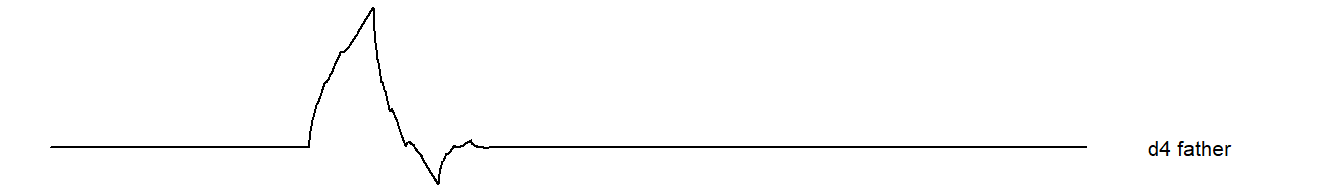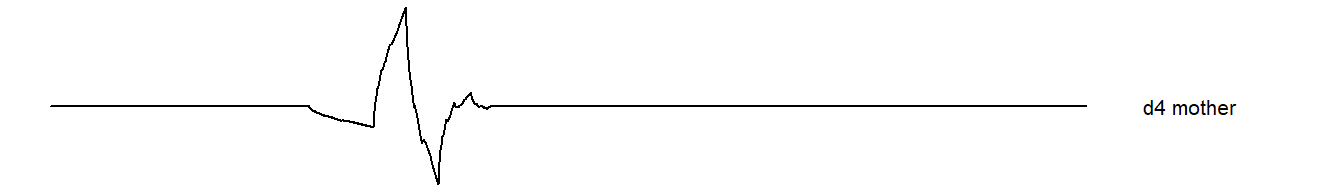You can shift the wavelets left and right.

{
graph_without_axis(calculate_father(2^9-2^7+1, 7),"d4 father\nshifted left")
graph_without_axis(calculate_father(2^9+2^7+1, 7),"d4 father\nshifted right")
graph_without_axis(calculate_mother(2^9-2^7+1, 7),"d4 mother\nshifted left")
graph_without_axis(calculate_mother(2^9+2^7+1, 7),"d4 mother\nshifted right")
}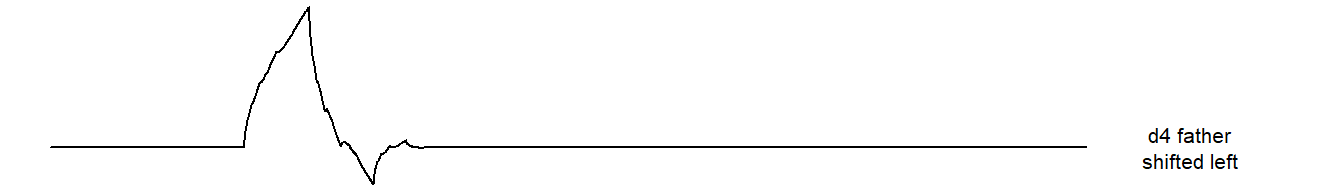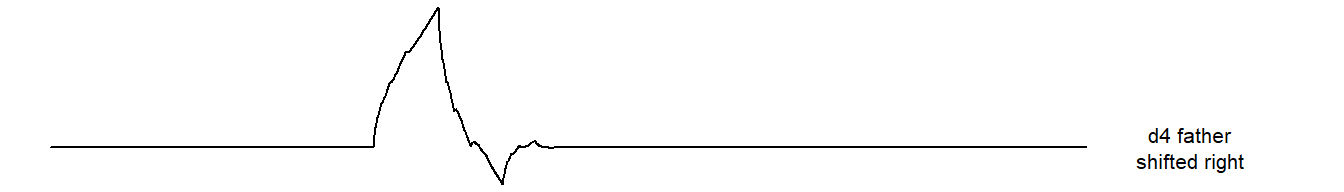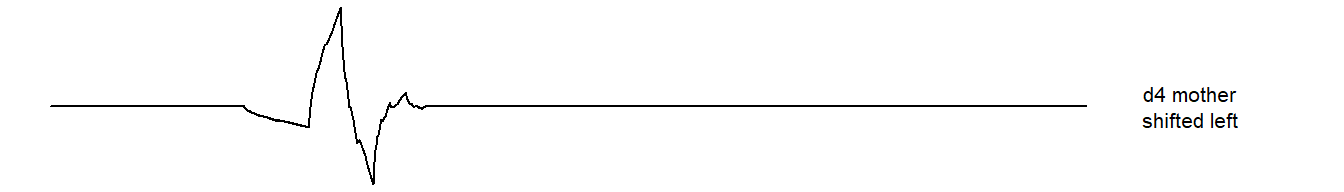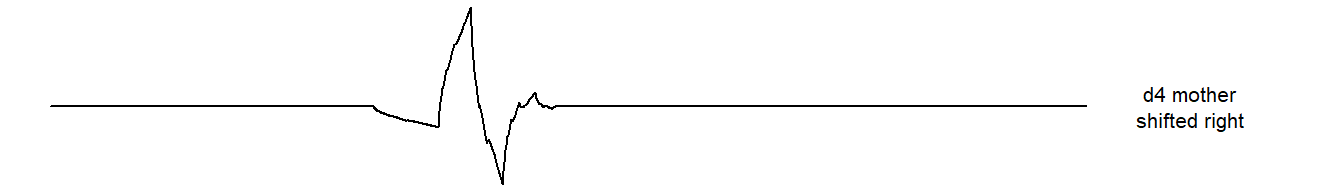When a wavelet crosses the boundary, there are several choices. The most common one is to wrap the wavelet around to the other side.

{
graph_without_axis(calculate_father(2^10+2^9+1+2^8+1, 7),"d4 father, wrapped")
graph_without_axis(calculate_mother(2^10+2^9+1+2^8+1, 7),"d4 mother, wrapped")
}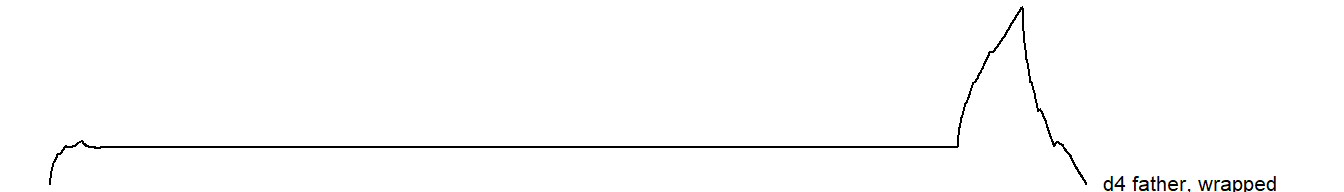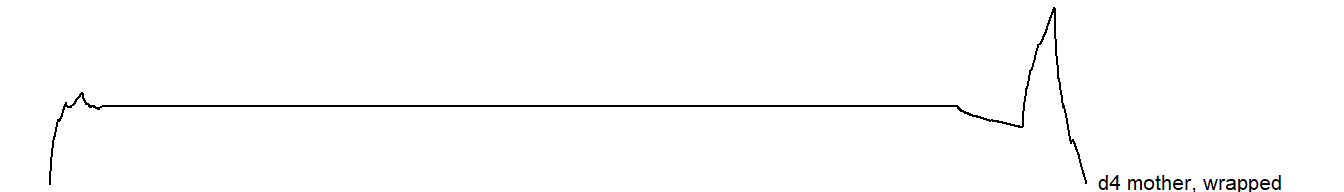You can stretch or squeeze the wavelets.

{
graph_without_axis(calculate_father(2^9+1, 8),"d4 father\nstretched")
graph_without_axis(calculate_father(2^9+1, 6),"d4 father\nsqueezed")
graph_without_axis(calculate_mother(2^9+1, 8),"d4 mother\nstretched")
graph_without_axis(calculate_mother(2^9+1, 6),"d4 mother\nsqueezed")
}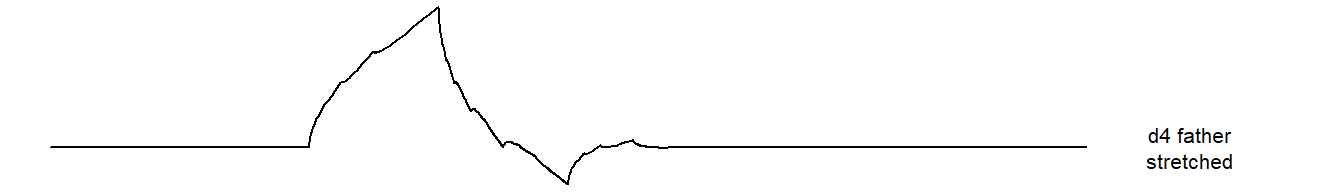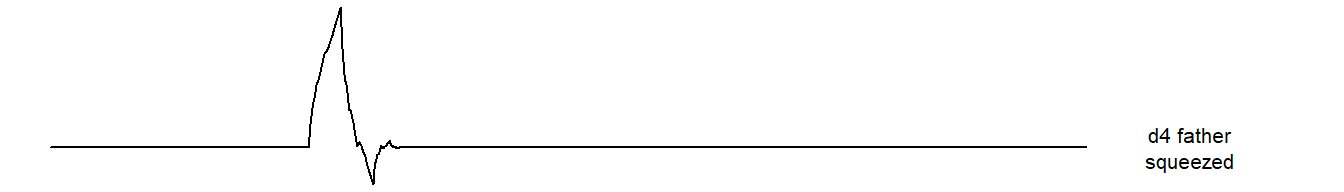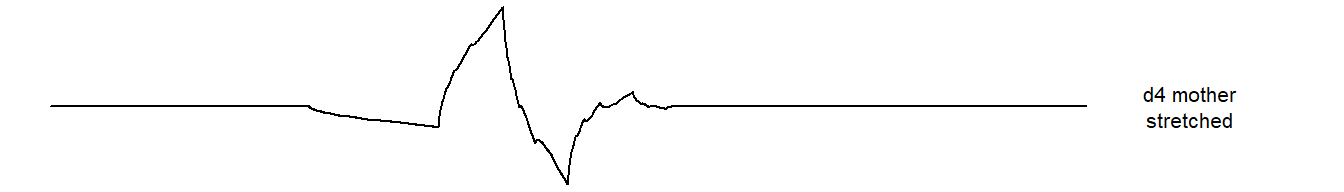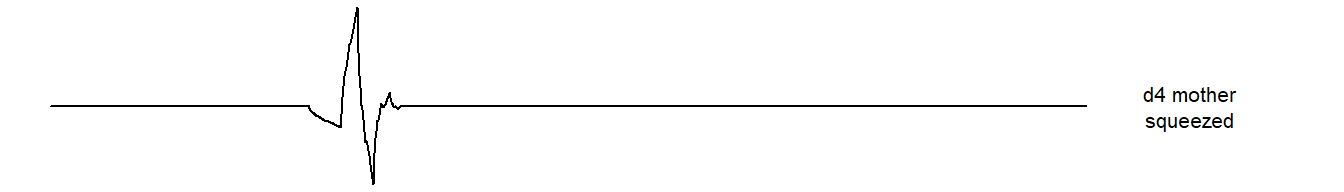Here are the father and mother wavelets at the most extreme.

{
graph_without_axis(calculate_father(2^9+1, 1),"d4 father\nmost extreme")
graph_without_axis(calculate_mother(2^9+1, 1),"d4 mother\nmost extreme")
}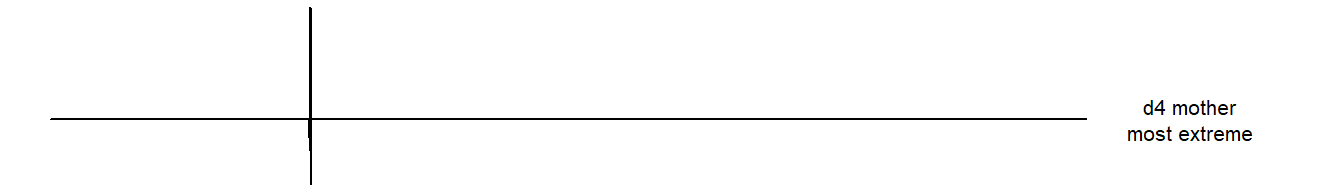Now, why the weird appearance? The d4 wavelet optimizes three properties simultaneously. The d4 wavelet chosen to have compact support, both for the wavelet decomposition and the inverse function that reconstructs the wavelet. The d4 wavelet is orthogonal both within a frequency band and across frequency bands. Finally, the d4 wavelet is orthogonal to any linear function, which simplifies the ability of the wavelet transform to isolate linear signals.

You don’t actually use the larger wavelets shown above. Instead you apply the most extreme wavelets in an iterative method that reduces the amount of data by half at each iteration. This makes the processing fast and easy. The most extreme D4 wavelets have only four coefficients.

g <- daubcqf(4)$h.0 g ##  0.4829629 0.8365163 0.2241439 -0.1294095 These coefficients represent the “high pass” filter. There is a complementary “low pass” filter that you get by reversing the coefficients and changing the sign of every other value. The term “high pass” means that it passes high frequencies above a certain cutoff and attenuates low frequencies. The term “low pass” means the opposite. Note that not all wavelets develop the low pass filter in the way described above. The construction is known as a quadrature mirror filter. There is an attractive simplicity to this approach. h <- -daubcqf(4)$h.1
h
##  -0.1294095 -0.2241439  0.8365163 -0.4829629

These two filters are orthonormal.

# The inline function %*% calculates a dot product of two vectors.
g %*% g
##      [,1]
## [1,]    1
h %*% h
##      [,1]
## [1,]    1
g %*% h
##              [,1]
## [1,] 6.938894e-18

The wavelet decomposition has an overlap, and (thankfully) the first half and the second half pieces are also orthogonal. Remember, orthogonality allows you to derive the mathematical properties of the wavelet decomposition easily and it preserves independence under certain settings.

g[1:2] %*% g[3:4]
##              [,1]
## [1,] 2.909201e-13
h[1:2] %*% h[3:4]
##              [,1]
## [1,] 2.909201e-13
g[1:2] %*% h[3:4]
##      [,1]
## [1,]    0
h[1:2] %*% g[3:4]
##      [,1]
## [1,]    0

The d4 filter also has one more nice property. The second filter is orthogonal to any linear signal

h %*% (1:4)
##               [,1]
## [1,] -1.000089e-12

This means that the linear portion of any signal is not filtered out, but is passed up the ladder of the decomposition. The d6 filter has six coefficients rather than four, and it does not filter out any linear or quadratic signal. The d8 filter has eight coefficients and it does not filter out any linear, quadratic, or cubic signal. In fact, it is this property involving polynomial signals that forms the basis of the Daubechies wavelets.

There’s one more property that is not immediately apparent from looking at the d4 wavelet. Some wavelets have simple forms for decomposition, but they get messier for the inverse of the decomposition. The d4 wavelet uses the same number of coefficients (4) in the inverse as it does in the composition. In fact, as you will see below, it uses the exact same vectors.

Let’s look at the first level of the wavelet decomposition.

For conveniece, split the decomposition into the left half (1:1024) and the right half (1025:2048).

Information about the highest frequency events are captured in W1 and information aobut the remainder of the signal are captured in V1. Notice how the largest absolute values in W1 are aligned with some of the very sharp spikes in the signal. The large absolute values in V1 correspond to some of the spikes in the signal that are not quite at the highest frequency as well as low frequency oscillations.

wt.1 <- mdwt(ecg, daubcqf(4)$h.0, L=1) V1 <- wt.1$y[   1:1024]
W1 <- wt.1$y[1025:2048] { needle_plot(V1, "V1", limits=range(ecg)) needle_plot(W1, "W1", limits=range(ecg)) graph_without_axis(ecg, "Original\nsignal", limits=range(ecg)) }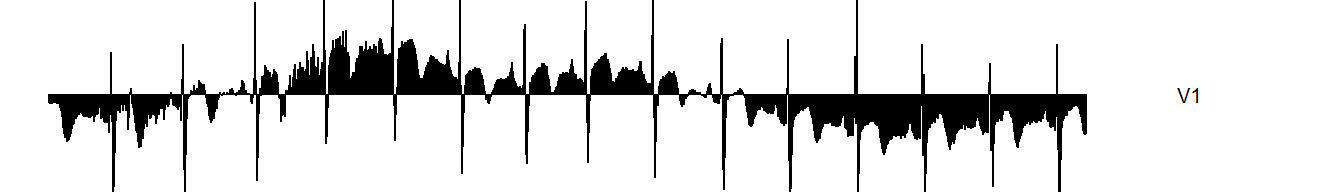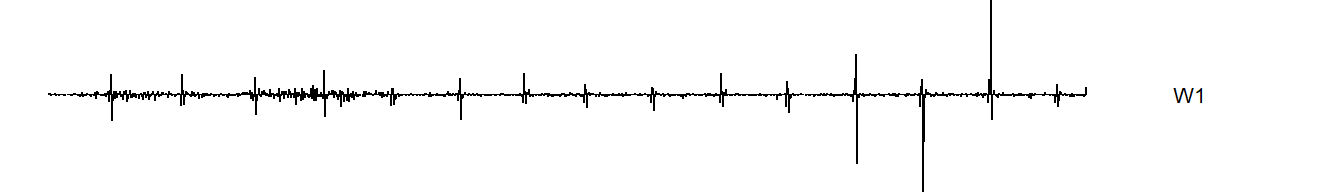The V1 coefficients represent the running dot product of the signal with the g vector. You skip every other data point in the running dot product (ignore the gray rows), and you rotate the dot product around to the end when reach the right hand side to avoid edge effects. The skipping past the second, fourth, sixth, etc. observation in the running dot product is described in some sources as down-sampling.Figure 1. G matrix The W1 coefficients represent the running dot product of the signal with the h vector, again with downsampling.Figure 2. H matrix c(V1[ 1], g %*% ecg[1:4]) ##  -0.1317145 -0.1317145 c(V1[ 2], g %*% ecg[3:6]) ##  -0.1399428 -0.1399428 c(V1[ 3], g %*% ecg[5:8]) ##  -0.148171 -0.148171 c(V1, g %*% ecg[2045:2048]) ##  -0.6455703 -0.6455703 c(V1, g %*% ecg[c(2047:2048, 1:2)]) ##  -0.6410026 -0.6410026 c(W1[ 1], h %*% ecg[1:4]) ##  0.02247964 0.02247964 c(W1[ 2], h %*% ecg[3:6]) ##  0.02468439 0.02468439 c(W1[ 3], h %*% ecg[5:8]) ##  -0.006023849 -0.006023849 c(W1, h %*% ecg[2045:2048]) ##  -0.007824638 -0.007824638 c(W1, h %*% ecg[c(2047:2048, 1:2)]) ##  0.12603 0.12603 Notice that you need to negate the h function to get the proper values for W. This is one of those scaling details in wavelets that will drive you nuts. A second pass applies the same pair of filters to V1. This creates 512 coefficients at the second highest frequency (W2) and 512 coefficients containing everything but W1 and W2 (V2). The last 1,024 coefficients are the same highest frequency values (W1) that were computed in the first pass. wt.2 <- mdwt(ecg, daubcqf(4)$h.0, L=2)
V2 <- wt.2$y[1:512] W2 <- wt.2$y[513:1024]
quality_check_W1 <- wt.2$y[1025:2048] all(quality_check_W1==W1) ##  TRUE { needle_plot(V2, "V2", limits=range(ecg)) needle_plot(W2, "W2", limits=range(ecg)) needle_plot(W1, "W1", limits=range(ecg)) graph_without_axis(ecg, "Original\nsignal", limits=range(ecg)) }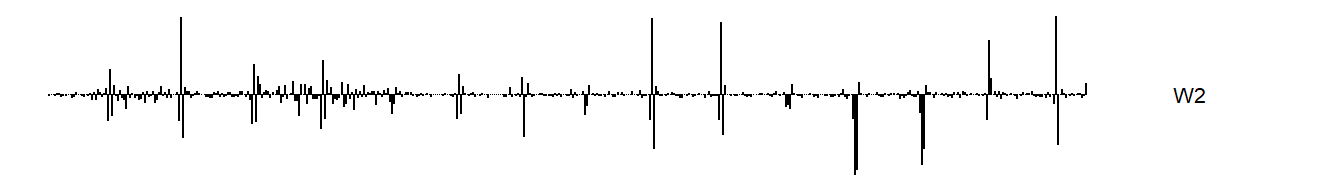c(V2[ 1], g %*% V1[1:4]) ##  -0.1961169 -0.1961169 c(V2[ 2], g %*% V1[3:6]) ##  -0.1989215 -0.1989215 c(V2[ 3], g %*% V1[5:8]) ##  -0.1863624 -0.1863624 c(V2, g %*% V1[1021:1024]) ##  -0.8719826 -0.8719826 c(V2, g %*% V1[c(1023:1024, 1:2)]) ##  -0.8594087 -0.8594087 c(W2[ 1], h %*% V1[1:4]) ##  -0.00920762 -0.00920762 c(W2[ 2], h %*% V1[3:6]) ##  0.003389415 0.003389415 c(W2[ 3], h %*% V1[5:8]) ##  -0.0003374554 -0.0003374554 c(W2, h %*% V1[1021:1024]) ##  -0.01334808 -0.01334808 c(W2, h %*% V1[c(1023:1024, 1:2)]) ##  0.1846255 0.1846255 You could get the same results as a level 2 wavelet transform by applying a level 1 wavelet transform to V1. quality_check <- mdwt(V1, daubcqf(4)$h.0, L=1)
quality_check_V2 <- quality_check$y[ 1: 512] quality_check_W2 <- quality_check$y[513:1024]
all(quality_check_V2==V2)
##  TRUE
all(quality_check_W2==W2)
##  TRUE

Note the order in which these are stored: 512 V2’s at the front, followed by 512 W2’s, and 1,024 W1’s bring up the rear. You don’t need to hang onto V1, because (as we will see in a minute), you can reconstruct the 1.024 V1’s from 512 V2’s and the 512 W2’s. Now, run the wavelet decomposition all the way up to level 3. This creates 256 V3’s, 256 W3’s, 512 W2’s, and 1,024 W1’s.

A third pass applies the same pair of filters to V2. This creates 256 coefficients at the third highest frequency (W3) and 256 coefficients containing information about all the lower frequency events (V3). These 512 values are followed by the 512 W2 coefficients and the 1,024 W3 coefficients.

wt.3 <- mdwt(ecg, daubcqf(4)$h.0, L=3) V3 <- wt.3$y[1:256]
W3 <- wt.3$y[257:512] W2_check3 <- wt.3$y[513:1024]
W1_check3 <- wt.3$y[1025:2048] all(W2_check3==W2) ##  TRUE all(W1_check3==W1) ##  TRUE { needle_plot(V3, "V3", limits=range(ecg)) needle_plot(W3, "W3", limits=range(ecg)) needle_plot(W2, "W2", limits=range(ecg)) needle_plot(W1, "W1", limits=range(ecg)) graph_without_axis(ecg, "Original\nsignal", limits=range(ecg)) }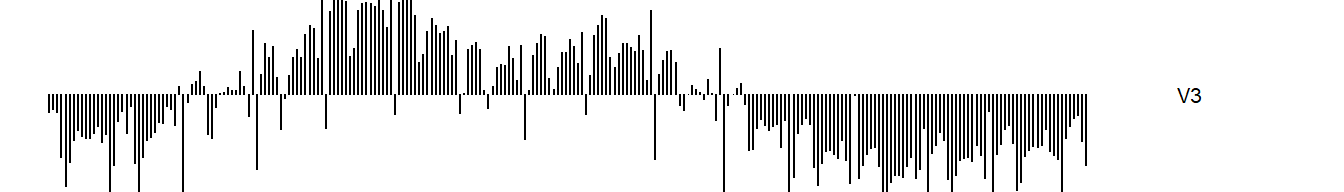Again, large coefficients in W3 are associated with more of the spikes in the original signal–spikes that are not quite as abrupt, perhaps, as the spikes captured in W1 and W2. The values of W3 and V3 are computed as before, but using V2 instead of V1 or the original signal. c(V3[ 1], g %*% V2[1:4]) ##  -0.28061 -0.28061 c(V3[ 2], g %*% V2[3:6]) ##  -0.2404948 -0.2404948 c(V3[ 3], g %*% V2[5:8]) ##  -0.2919882 -0.2919882 c(V3, g %*% V2[509:512]) ##  -0.7727788 -0.7727788 c(V3, g %*% V2[c(511:512, 1:2)]) ##  -1.158261 -1.158261 c(W3[ 1], h %*% V2[1:4]) ##  -0.002777659 -0.002777659 c(W3[ 2], h %*% V2[3:6]) ##  0.03857375 0.03857375 c(W3[ 3], h %*% V2[5:8]) ##  0.0963033 0.0963033 c(W3, h %*% V2[509:512]) ##  -0.1298701 -0.1298701 c(W3, h %*% V2[c(511:512, 1:2)]) ##  0.2374907 0.2374907 Here’s an outline of how the wavelet transform works, level by level. Take the original 2,048 signal values and transform them into 1.024 very high frequency values (W1) and what’s left over (V1). Take the 1,024 V1 values and split them into 512 high frequency values (W2) and what’s left over (V2). Take those V2 values and split them. Keep on spliting until there are only a handful of V values left. I won’t show the details of further calculation, but here is what the graph looks like. wt.9 <- mdwt(ecg, daubcqf(4)$h.0, L=9)
V9 <- wt.9$y[ 1: 4] W9 <- wt.9$y[   5:   8]
W8 <- wt.9$y[ 9: 16] W7 <- wt.9$y[  17:  32]
W6 <- wt.9$y[ 33: 64] W5 <- wt.9$y[  65: 128]
W4 <- wt.9$y[ 129: 256] W3 <- wt.9$y[ 257: 512]
W2 <- wt.9$y[ 513:1024] W1 <- wt.9$y[1025:2048]
{
needle_plot(V9, "V9", limits=range(ecg))
needle_plot(W9, "W9", limits=range(ecg))
needle_plot(W8, "W8", limits=range(ecg))
needle_plot(W7, "W7", limits=range(ecg))
needle_plot(W6, "W6", limits=range(ecg))
needle_plot(W5, "W5", limits=range(ecg))
needle_plot(W4, "W4", limits=range(ecg))
needle_plot(W3, "W3", limits=range(ecg))
needle_plot(W2, "W2", limits=range(ecg))
needle_plot(W1, "W1", limits=range(ecg))
graph_without_axis(ecg, "Original\nsignal", limits=range(ecg))
}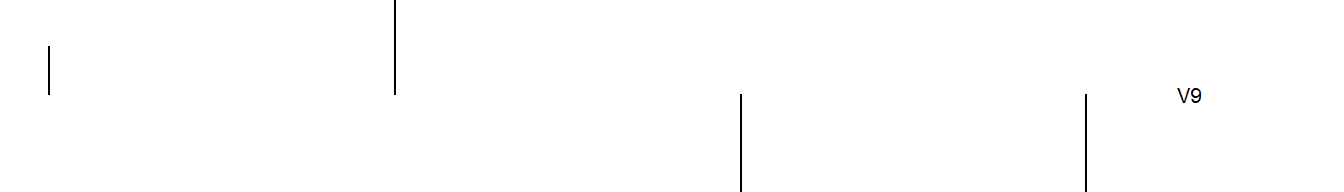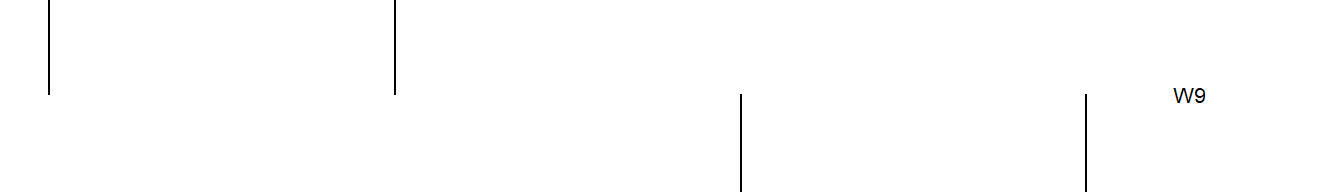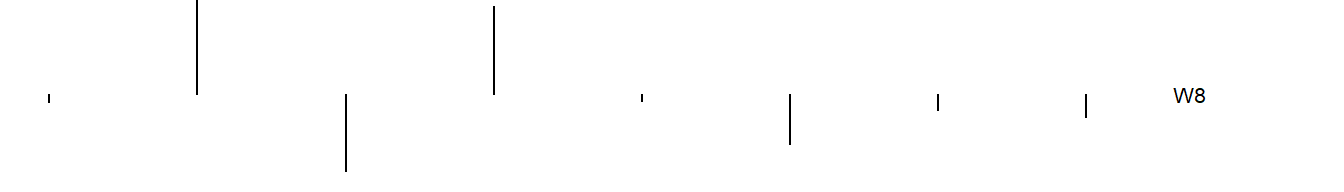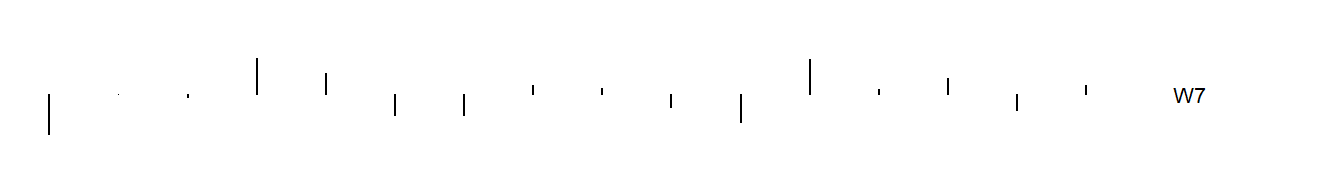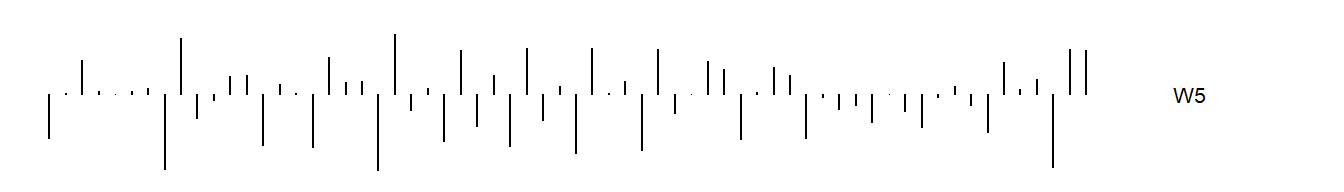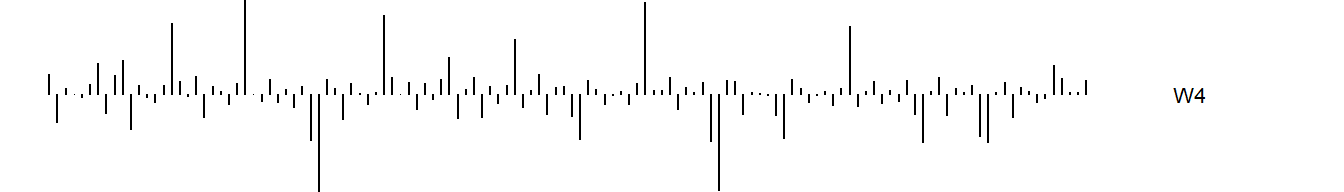### Reconstruction of the original signal

The best explanation of the inverse wavelet transformation is a page buried deep in Ian Kaplan’s website, bearcave. Also helpful is this pdf file of Chapter 2 of a book on wavelets.

Reconstruction of the original signal works in a similar fashion.

First you dilute the W and V values at the top of the pyramid. Then you compute a running dot product of the reversed g vector with the diluted V9 values plus a running dot product of the reversed h vector with the diluted W9 values. Please note that using the reversed order for the g and h vectors is unique to the Daubechies family of wavelets. Other wavelets will use an entirely different set of vectors in the rolling dot product.Figure 3. Matrix representation of reconstruction

V9_diluted <- rep(0, 8)
V9_diluted[(1:4)*2-1] <- V9
W9_diluted <- rep(0, 8)
W9_diluted[(1:4)*2-1] <- W9

wt.8 <- mdwt(ecg, daubcqf(4)$h.0, L=8) V8 <- wt.8$y[1:8]

c(V8, rev(g) %*% V9_diluted[c(6, 7, 8, 1)] + rev(h) %*% W9_diluted[c(6, 7, 8, 1)])
##  -3.900132 -3.900132
c(V8, rev(g) %*% V9_diluted[c(7, 8, 1, 2)] + rev(h) %*% W9_diluted[c(7, 8, 1, 2)])
##  1.91968 1.91968
c(V8, rev(g) %*% V9_diluted[c(8, 1, 2, 3)] + rev(h) %*% W9_diluted[c(8, 1, 2, 3)])
##  6.640577 6.640577
c(V8, rev(g) %*% V9_diluted[c(1, 2, 3, 4)] + rev(h) %*% W9_diluted[c(1, 2, 3, 4)])
##  3.192375 3.192375
c(V8, rev(g) %*% V9_diluted[c(2, 3, 4, 5)] + rev(h) %*% W9_diluted[c(2, 3, 4, 5)])
##  2.59452 2.59452
c(V8, rev(g) %*% V9_diluted[c(3, 4, 5, 6)] + rev(h) %*% W9_diluted[c(3, 4, 5, 6)])
##  -4.018609 -4.018609
c(V8, rev(g) %*% V9_diluted[c(4, 5, 6, 7)] + rev(h) %*% W9_diluted[c(4, 5, 6, 7)])
##  -6.601313 -6.601313
c(V8, rev(g) %*% V9_diluted[c(5, 6, 7, 8)] + rev(h) %*% W9_diluted[c(5, 6, 7, 8)])
##  -5.057149 -5.057149

To move from V8 to V7, dilute the V8 and W8 vectors and apply a running dot product again.

V8_diluted <- rep(0, 16)
V8_diluted[(1:8)*2-1] <- V8
W8_diluted <- rep(0, 16)
W8_diluted[(1:8)*2-1] <- W8

wt.7 <- mdwt(ecg, daubcqf(4)$h.0, L=7) V7 <- wt.7$y[1:16]

c(V7[ 1], rev(g) %*% V8_diluted[c(14, 15, 16,  1)] + rev(h) %*% W8_diluted[c(14, 15, 16,  1)])
##  -3.315508 -3.315508
c(V7[ 2], rev(g) %*% V8_diluted[c(15, 16,  1,  2)] + rev(h) %*% W8_diluted[c(15, 16,  1,  2)])
##  -2.398146 -2.398146
c(V7[ 3], rev(g) %*% V8_diluted[c(16,  1,  2,  3)] + rev(h) %*% W8_diluted[c(16,  1,  2,  3)])
##  -0.2769286 -0.2769286
c(V7[ 4], rev(g) %*% V8_diluted[c( 1,  2,  3,  4)] + rev(h) %*% W8_diluted[c( 1,  2,  3,  4)])
##  1.782748 1.782748
c(V7, rev(g) %*% V8_diluted[c(13, 14, 15, 16)] + rev(h) %*% W8_diluted[c(12, 13, 14, 15)])
##  -3.171176 -3.536357

Here is the reconstruction of V6

V7_diluted <- rep(0, 32)
V7_diluted[(1:16)*2-1] <- V7
W7_diluted <- rep(0, 32)
W7_diluted[(1:16)*2-1] <- W7

wt.6 <- mdwt(ecg, daubcqf(4)$h.0, L=6) V6 <- wt.6$y[1:32]

c(V6[ 1], rev(g) %*% V7_diluted[c(30, 31, 32,  1)] + rev(h) %*% W7_diluted[c(30, 31, 32,  1)])
##  -2.096562 -2.096562
c(V6[ 2], rev(g) %*% V7_diluted[c(31, 32,  1,  2)] + rev(h) %*% W7_diluted[c(31, 32,  1,  2)])
##  -2.294492 -2.294492
c(V6[ 3], rev(g) %*% V7_diluted[c(32,  1,  2,  3)] + rev(h) %*% W7_diluted[c(32,  1,  2,  3)])
##  -2.441797 -2.441797
c(V6[ 4], rev(g) %*% V7_diluted[c( 1,  2,  3,  4)] + rev(h) %*% W7_diluted[c( 1,  2,  3,  4)])
##  -1.265345 -1.265345
c(V6, rev(g) %*% V7_diluted[c(29, 30, 31, 32)] + rev(h) %*% W7_diluted[c(29, 30, 31, 32)])
##  -2.033527 -2.033527

The process for reconstructing V5, V4, etc. is similar. At the final step, dilute V1 and W1. The running dot product will recreate the original signal.

What exactly do V9, V8, V6, etc. measure? They are coefficients associated with the mother wavelet and reconstruct a signal with high frequency events removed.

V9_coefficients <- rep(0, 2048)
V9_coefficients[1:4] <- V9
V9_signal <- as.vector(midwt(V9_coefficients, daubcqf(4)$h.0, L=9)$x)

V8_coefficients <- rep(0, 2048)
V8_coefficients[1:8] <- V8
V8_signal <- as.vector(midwt(V8_coefficients, daubcqf(4)$h.0, L=8)$x)

V7_coefficients <- rep(0, 2048)
V7_coefficients[1:16] <- V7
V7_signal <- as.vector(midwt(V7_coefficients, daubcqf(4)$h.0, L=7)$x)

V6_coefficients <- rep(0, 2048)
V6_coefficients[1:32] <- V6
V6_signal <- as.vector(midwt(V6_coefficients, daubcqf(4)$h.0, L=6)$x)

wt.5 <- mdwt(ecg, daubcqf(4)$h.0, L=5) V5 <- wt.5$y[1:64]
V5_coefficients <- rep(0, 2048)
V5_coefficients[1:64] <- V5
V5_signal <- as.vector(midwt(V5_coefficients, daubcqf(4)$h.0, L=5)$x)

wt.4 <- mdwt(ecg, daubcqf(4)$h.0, L=4) V4 <- wt.4$y[1:128]
V4_coefficients <- rep(0, 2048)
V4_coefficients[1:128] <- V4
V4_signal <- as.vector(midwt(V4_coefficients, daubcqf(4)$h.0, L=4)$x)

wt.3 <- mdwt(ecg, daubcqf(4)$h.0, L=3) V3 <- wt.3$y[1:256]
V3_coefficients <- rep(0, 2048)
V3_coefficients[1:256] <- V3
V3_signal <- as.vector(midwt(V3_coefficients, daubcqf(4)$h.0, L=3)$x)

wt.2 <- mdwt(ecg, daubcqf(4)$h.0, L=2) V2 <- wt.2$y[1:512]
V2_coefficients <- rep(0, 2048)
V2_coefficients[1:512] <- V2
V2_signal <- as.vector(midwt(V2_coefficients, daubcqf(4)$h.0, L=2)$x)

wt.1 <- mdwt(ecg, daubcqf(4)$h.0, L=1) V1 <- wt.1$y[1:1024]
V1_coefficients <- rep(0, 2048)
V1_coefficients[1:1024] <- V1
V1_signal <- as.vector(midwt(V1_coefficients, daubcqf(4)$h.0, L=1)$x)

{
graph_without_axis(V9_signal, "V9", limits=range(ecg))
graph_without_axis(V8_signal, "V8", limits=range(ecg))
graph_without_axis(V7_signal, "V7", limits=range(ecg))
graph_without_axis(V6_signal, "V6", limits=range(ecg))
graph_without_axis(V5_signal, "V5", limits=range(ecg))
graph_without_axis(V4_signal, "V4", limits=range(ecg))
graph_without_axis(V3_signal, "V3", limits=range(ecg))
graph_without_axis(V2_signal, "V2", limits=range(ecg))
graph_without_axis(V1_signal, "V1", limits=range(ecg))
graph_without_axis(ecg, "Original\nsignal", limits=range(ecg))
}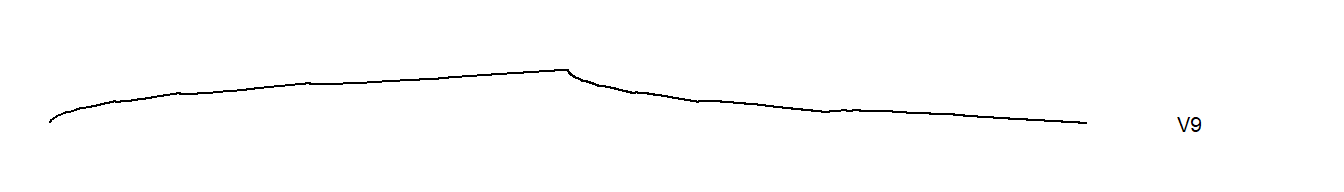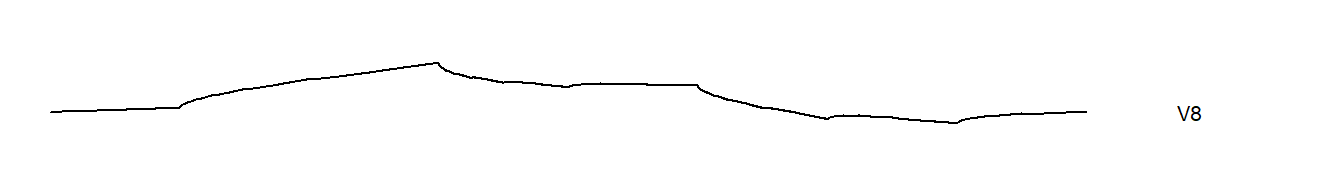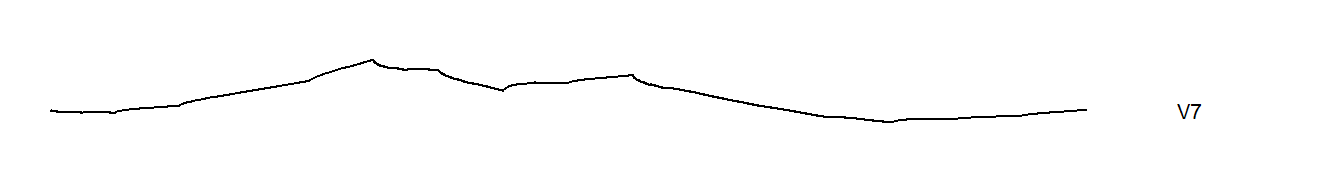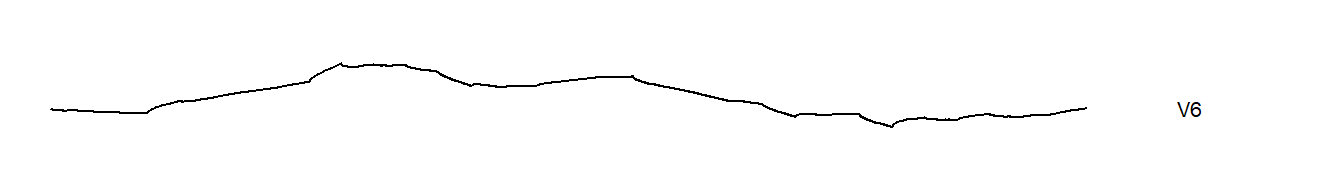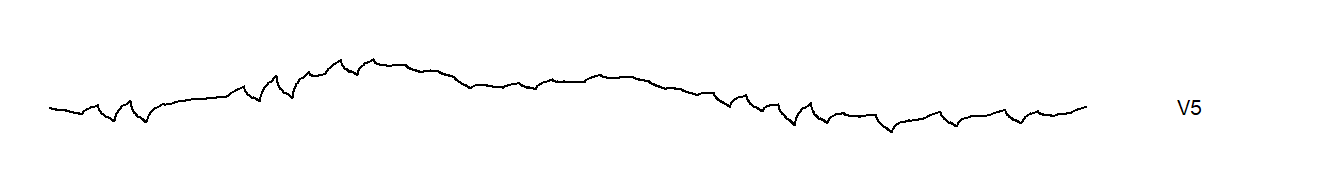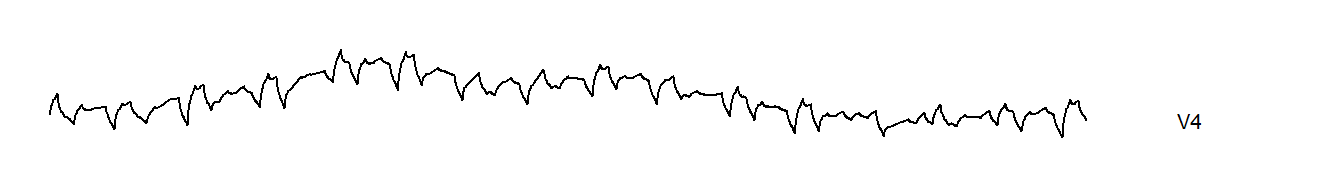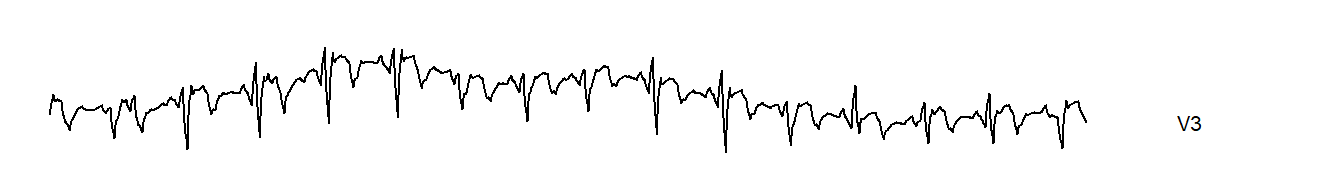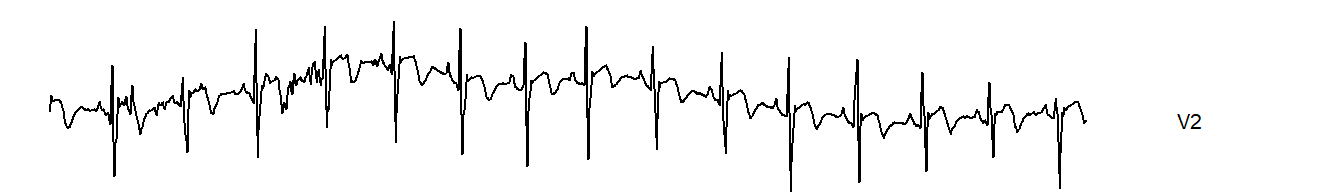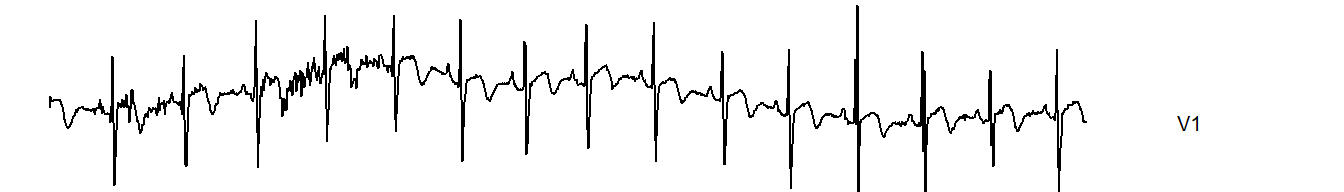What exactly do W9, W8, W7, etc. measure? They are coefficients associated with the father wavelet and reconstruct a signal with at each frequency level.

W9_coefficients <- rep(0, 2048)
W9_coefficients[5:8] <- W9
W9_signal <- as.vector(midwt(W9_coefficients, daubcqf(4)$h.0, L=9)$x)

W8_coefficients <- rep(0, 2048)
W8_coefficients[9:16] <- W8
W8_signal <- as.vector(midwt(W8_coefficients, daubcqf(4)$h.0, L=9)$x)

W7_coefficients <- rep(0, 2048)
W7_coefficients[17:32] <- W7
W7_signal <- as.vector(midwt(W7_coefficients, daubcqf(4)$h.0, L=9)$x)

W6_coefficients <- rep(0, 2048)
W6_coefficients[33:64] <- W6
W6_signal <- as.vector(midwt(W6_coefficients, daubcqf(4)$h.0, L=9)$x)

W5_coefficients <- rep(0, 2048)
W5_coefficients[65:128] <- W5
W5_signal <- as.vector(midwt(W5_coefficients, daubcqf(4)$h.0, L=9)$x)

W4_coefficients <- rep(0, 2048)
W4_coefficients[129:256] <- W4
W4_signal <- as.vector(midwt(W4_coefficients, daubcqf(4)$h.0, L=9)$x)

W3_coefficients <- rep(0, 2048)
W3_coefficients[257:512] <- W3
W3_signal <- as.vector(midwt(W3_coefficients, daubcqf(4)$h.0, L=9)$x)

W2_coefficients <- rep(0, 2048)
W2_coefficients[513:1024] <- W2
W2_signal <- as.vector(midwt(W2_coefficients, daubcqf(4)$h.0, L=9)$x)

W1_coefficients <- rep(0, 2048)
W1_coefficients[1025:2048] <- W1
W1_signal <- as.vector(midwt(W1_coefficients, daubcqf(4)$h.0, L=9)$x)

{
graph_without_axis(W9_signal, "W9", limits=range(ecg))
graph_without_axis(W8_signal, "W8", limits=range(ecg))
graph_without_axis(W7_signal, "W7", limits=range(ecg))
graph_without_axis(W6_signal, "W6", limits=range(ecg))
graph_without_axis(W5_signal, "W5", limits=range(ecg))
graph_without_axis(W4_signal, "W4", limits=range(ecg))
graph_without_axis(W3_signal, "W3", limits=range(ecg))
graph_without_axis(W2_signal, "W2", limits=range(ecg))
graph_without_axis(W1_signal, "W1", limits=range(ecg))
graph_without_axis(ecg, "Original\nsignal", limits=range(ecg))
}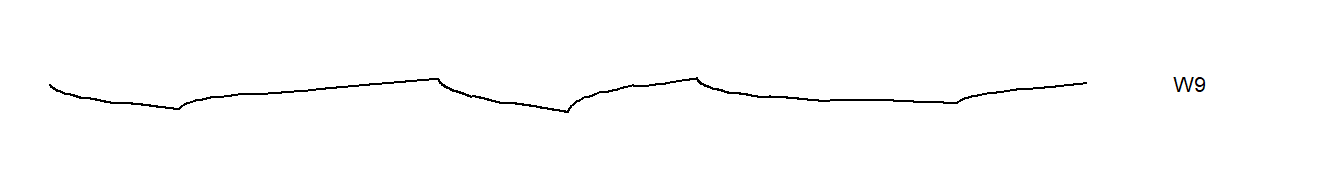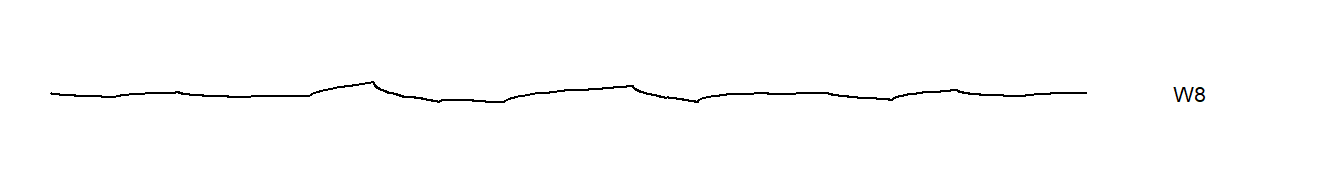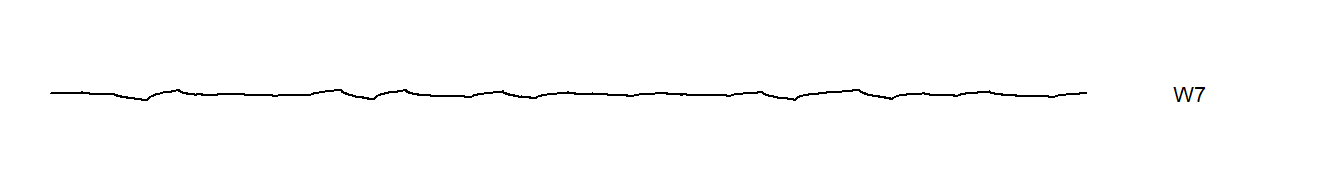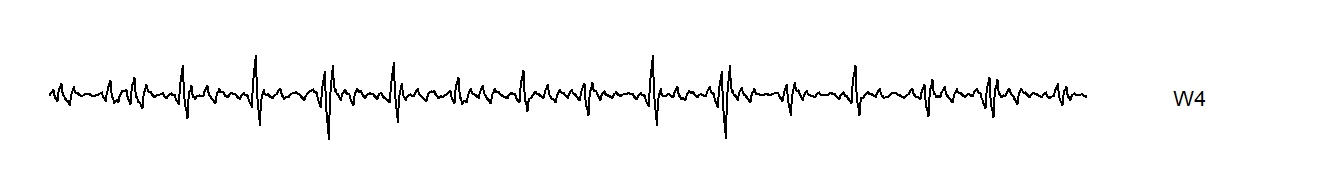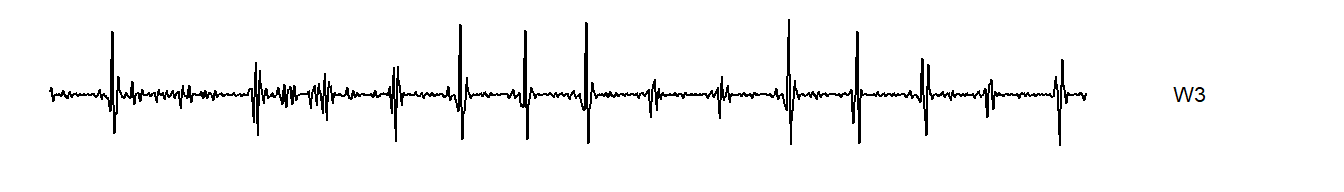### Two dimensional wavelets

There are simple generalizations of wavelets for two dimensional images. Here’s a simple image, which represents a 256 by 256 bit grayscale image of a dog.

knitr::opts_chunk$set(fig.width=5, fig.height=5) fn <- "https://www2.isye.gatech.edu/isyebayes/data/klaus256.asc" img <- read.table(fn) img <- 2*img-1 img.plot <- function(imat,n=256) { xsq <- c(0,0,1,1) ysq <- c(0,1,1,0) par(mar=rep(0,4),xaxs="i",yaxs="i") plot(c(1,n+1),c(1,n+1),type="n",axes=FALSE) # imat <- imat/max(abs(imat)) for (i in 1:n) { for (j in 1:n){ d <- 0.5*imat[n+1-j,i]+0.5 d <- min(1,max(0,d)) polygon(xsq+i,ysq+j,density=-1,border=NA,col=rgb(d,d,d)) } } } img.plot(img)Let’s also move to a slightly lengthier wavelet, the d8 wavelet. g <- daubcqf(8)$h.0
g
##   0.23037781  0.71484657  0.63088077 -0.02798377 -0.18703481  0.03084138
##   0.03288301 -0.01059740
h <- -daubcqf(8)$h.1 h ##  -0.01059740 -0.03288301 0.03084138 0.18703481 -0.02798377 -0.63088077 ##  0.71484657 -0.23037781 The d8 wavelet is a bit smoother than d4. It passes linear, quadratic, and cubic functions through to the top of the pyramid, unlike the d4 wavelet which only passes linear functions through. There is slightly greater computational complexity and edge effects can be a bit more pronounced, but these are minor issues. In one dimension, the d8 wavelet looks like this. d8_father_coefficients <- rep(0, 2048) d8_father_coefficients <- 1 d8_father <- as.vector(midwt(d8_father_coefficients, daubcqf(8)$h.0, L=8)$x) d8_mother_coefficients <- rep(0, 2048) d8_mother_coefficients <- 1 d8_mother <- as.vector(midwt(d8_mother_coefficients, daubcqf(8)$h.0, L=8)$x) { graph_without_axis(d8_father, "D8 father") graph_without_axis(d8_mother, "D8 mother") }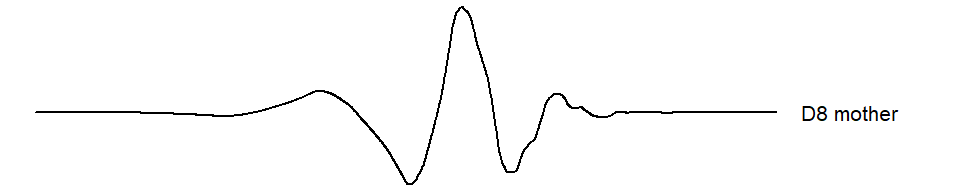For a two dimensional image, you take the outer product of g with itself to get an 8 by 8 matrix. This matrix is then slid around on the image to get the V1 coefficients. The W1 coefficents are produced by a similar set of three outer products: the outer product of h with g, the outer product of g with h and the outer product of h with h. wt.img.4 <- mdwt(img, daubcqf(8)$h.0, L=4)
reconstructed.img <- midwt(wt.img.4$y, daubcqf(8)$h.0, L=4)
img.plot(reconstructed.img$x)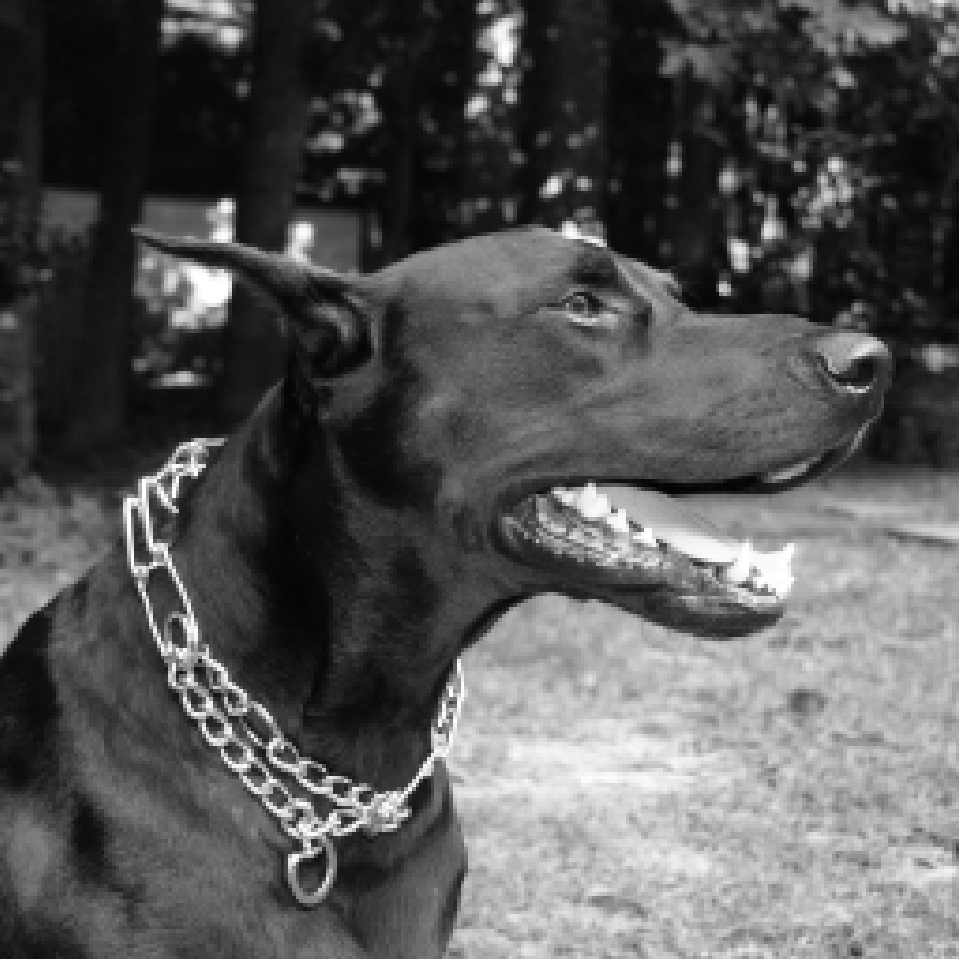wt.img.1 <- mdwt(img, daubcqf(8)$h.0, L=1)
c(sum(img[1:8,1:8]*outer(g,g)), wt.img.1$y[ 1, 1]) ##  -1.580973 -1.580973 c(sum(img[1:8,1:8]*outer(g,h)),wt.img.1$y[  1, 129])
##  -0.005042734 -0.005042734
c(sum(img[1:8,1:8]*outer(h,g)),wt.img.1$y[129, 1]) ##  -0.01225749 -0.01225749 c(sum(img[1:8,1:8]*outer(h,h)),wt.img.1$y[129, 129])
##  -0.002825861 -0.002825861

The first level of the 2D wavelet decomposition for a 256256 image produces 128128 V1 coefficients and 3128128 W1 coefficients. The second level produces 6464 V2 coefficients and 36464 W2 coefficients. If you continue this to the sixth level, you get 44 V6 coefficients and 344 W6 coefficients. This continues until the number of V coefficients becomes small. This is a pyrimidal cascade again, but this time a cascade in two dimensions.

### Wavelet coefficients arrangement

Now there are three different sets of W’s, one associated with outer(h,g), one with outer(g,h), and one with outer(h,h). The first represents high frequency changes in the horizontal direction and the second represents high frequency changes in the vertical direction. The third represents high frequency changes in a diagonal direction. In an image, a high frequency event represents a sharp transition between light to dark or dark to light. These transitions frequently represent boundaries or edges in an image.

x <- c(700, 300, 700)
y <- c(700, 300, 300)
m <- c(
"W1\nh %*% g",
"W1\ng %*% h",
"W1\nh %*% h"
)
data.frame(x, y, m) %>%
ggplot(aes(x, y, label=m)) +
expand_limits(x=c(100, 900), y=c(100, 900)) +
geom_segment(x=100, y=100, xend=900, yend=100) +
geom_segment(x=900, y=100, xend=900, yend=900) +
geom_segment(x=900, y=900, xend=100, yend=900) +
geom_segment(x=100, y=900, xend=100, yend=100) +
geom_segment(x=500, y=100, xend=500, yend=900) +
geom_segment(x=100, y=500, xend=900, yend=500) +
theme_void() +
geom_text() -> layout_1
layout_1 +
geom_text(x=300, y=700, label="V1\ng %*% g")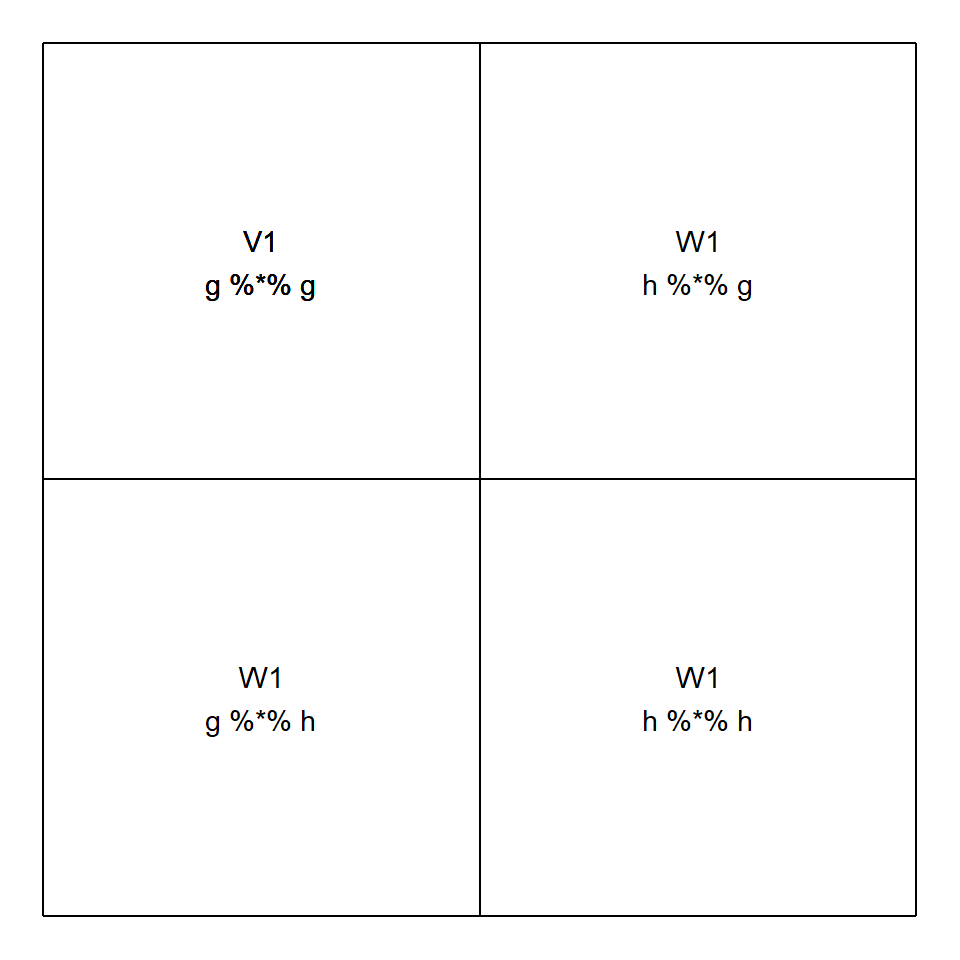layout_1+
geom_segment(x=300, y=500, xend=300, yend=900) +
geom_segment(x=100, y=700, xend=500, yend=700) +
geom_text(x=400, y=800, label="W2\nh %*% g") +
geom_text(x=200, y=600, label="W2\ng %*% h") +
geom_text(x=400, y=600, label="W2\nh %*% h") -> layout_2
layout_2 +
geom_text(x=200, y=800, label="V2\ng %*% g")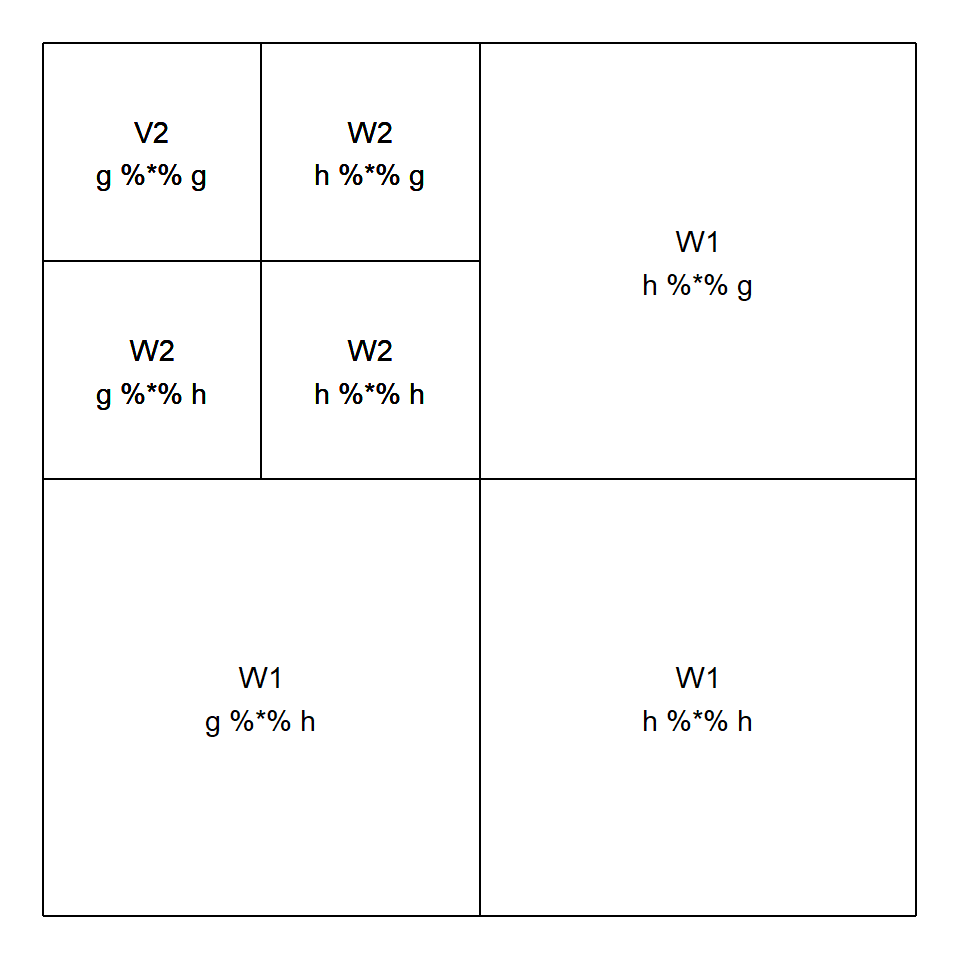layout_2 +
geom_segment(x=200, y=700, xend=200, yend=900) +
geom_segment(x=100, y=800, xend=300, yend=800) +
geom_text(x=250, y=850, label="W3") +
geom_text(x=150, y=750, label="W3") +
geom_text(x=250, y=750, label="W3") +
geom_text(x=150, y=850, label="etc.")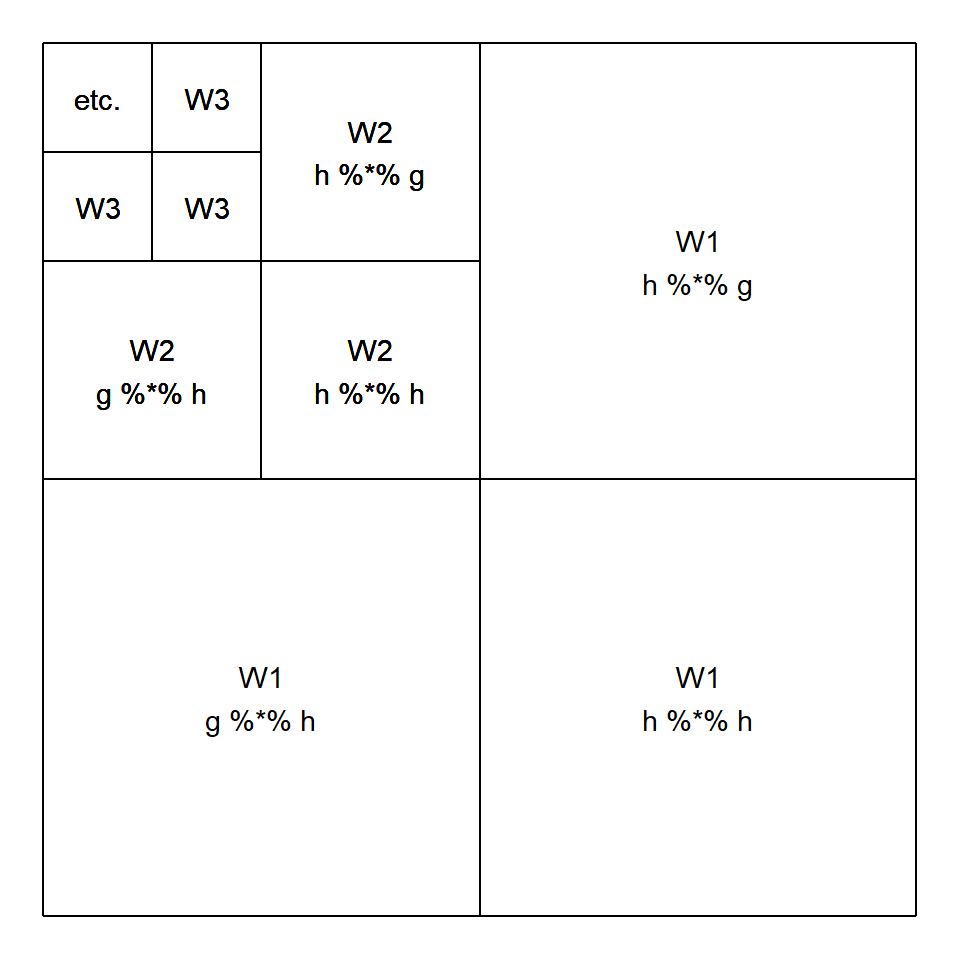gg1 <- matrix(0, nrow=256, ncol=256)
gg1[1:128, 1:128] <- wt.img.1$y[1:128, 1:128] gg1_signal <- midwt(gg1, daubcqf(8)$h.0, L=1)
img.plot(gg1_signal$x)gh1 <- matrix(0, nrow=256, ncol=256) gh1[1:128, 129:256] <- wt.img.1$y[1:128, 129:256]
gh1_signal <- midwt(gh1, daubcqf(8)$h.0, L=1) img.plot(gh1_signal$x)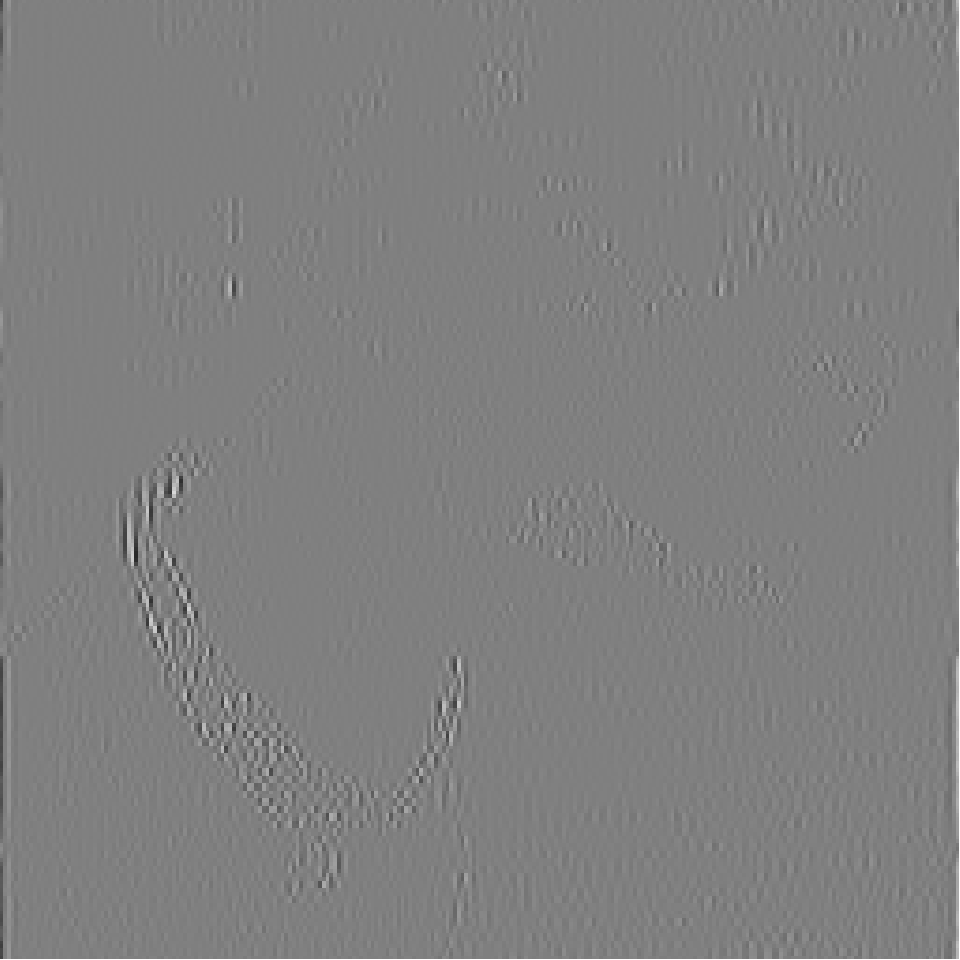hg1 <- matrix(0, nrow=256, ncol=256)
hg1[1:128, 129:256] <- wt.img.1$y[129:256, 1:128] hg1_signal <- midwt(hg1, daubcqf(8)$h.0, L=1)
img.plot(hg1_signal$x)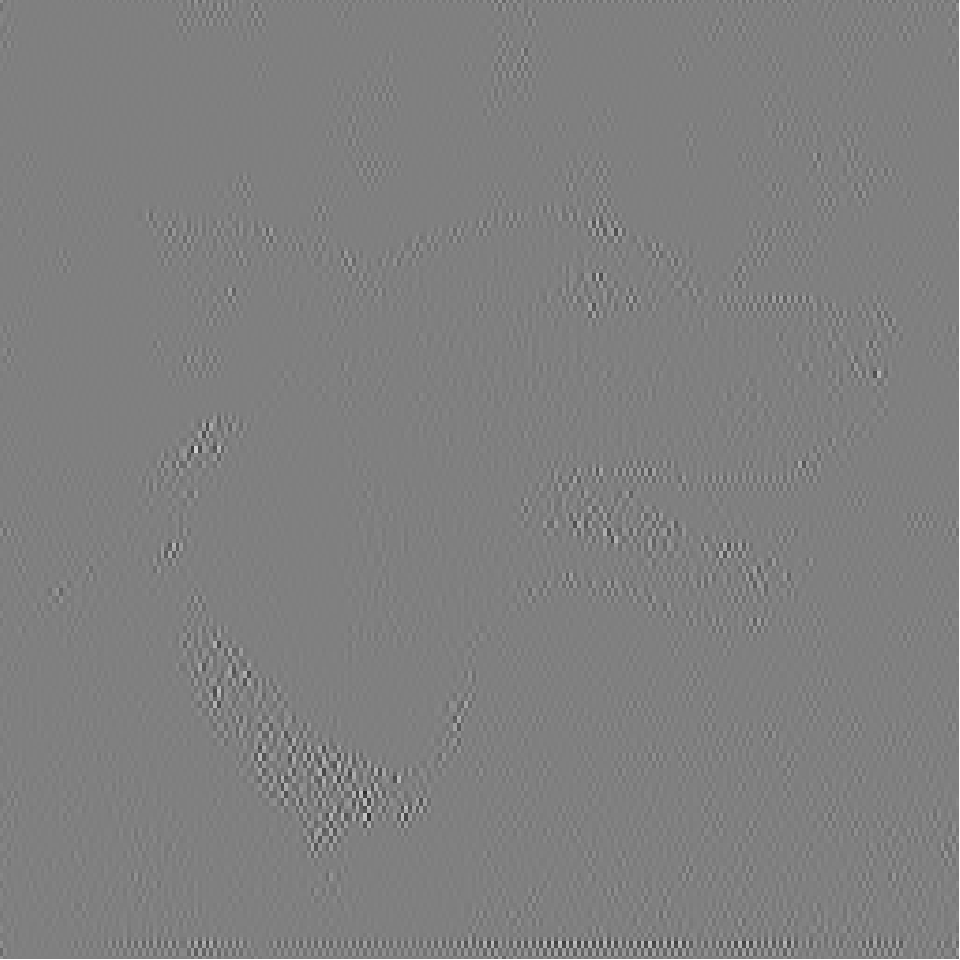hh1 <- matrix(0, nrow=256, ncol=256) hh1[129:256, 129:256] <- wt.img.1$y[129:256, 129:256]
hh1_signal <- midwt(hh1, daubcqf(8)$h.1, L=1) img.plot(hh1_signal$x)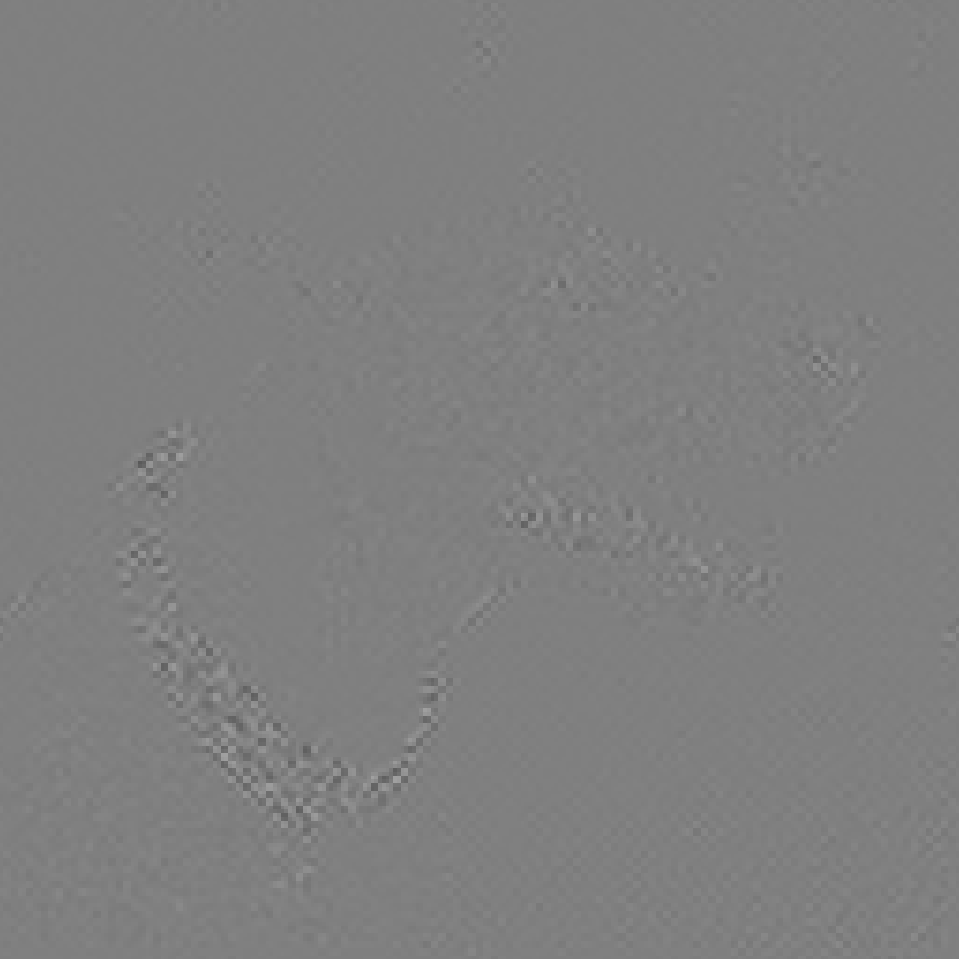gg4 <- matrix(0, nrow=256, ncol=256)
gg4[1:16, 1:16] <- wt.img.4$y[1:16, 1:16] gg4_signal <- midwt(gg4, daubcqf(8)$h.0, L=4)
img.plot(gg4_signal$x)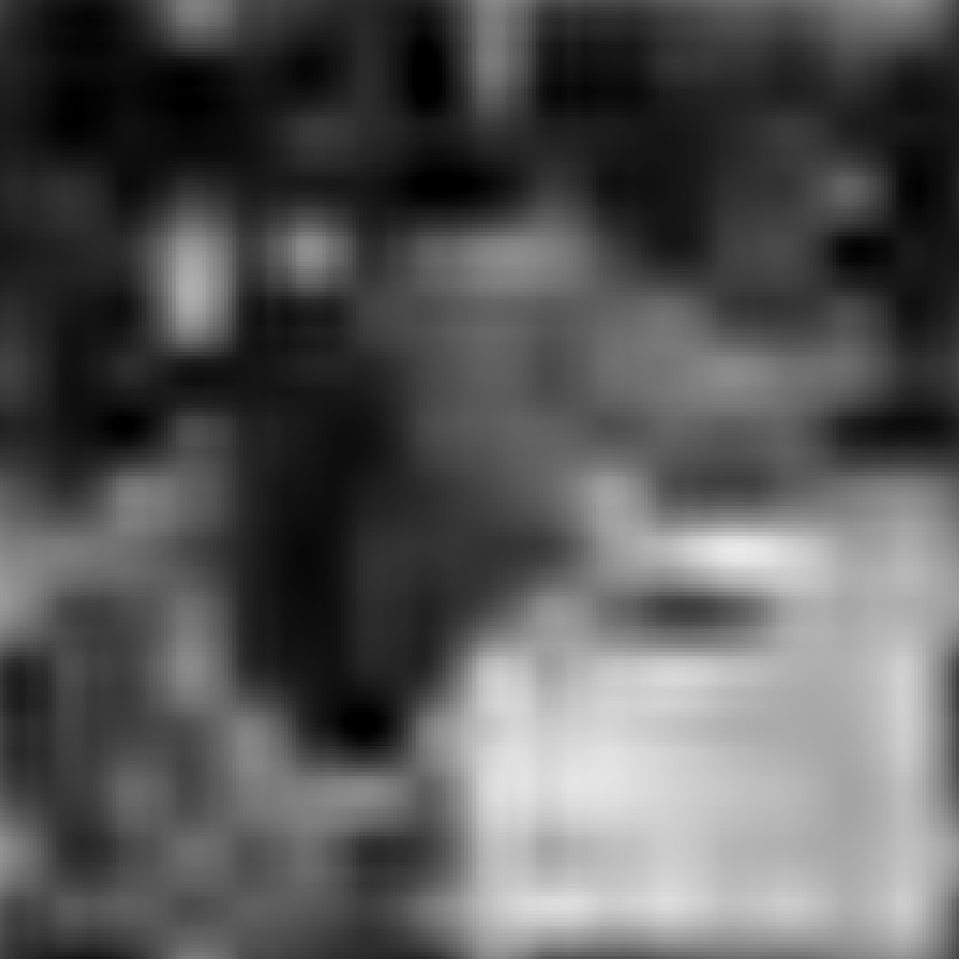gh4 <- matrix(0, nrow=256, ncol=256) gh4[1:16, 17:32] <- wt.img.4$y[1:16, 17:32]
gh4[1:32, 33:64] <- wt.img.4$y[1:32, 33:64] gh4[1:64, 65:128] <- wt.img.4$y[1:64, 65:128]
gh4[1:128,129:256] <- wt.img.4$y[1:128, 129:256] gh4_signal <- midwt(gh4, daubcqf(8)$h.0, L=4)
img.plot(gh4_signal$x)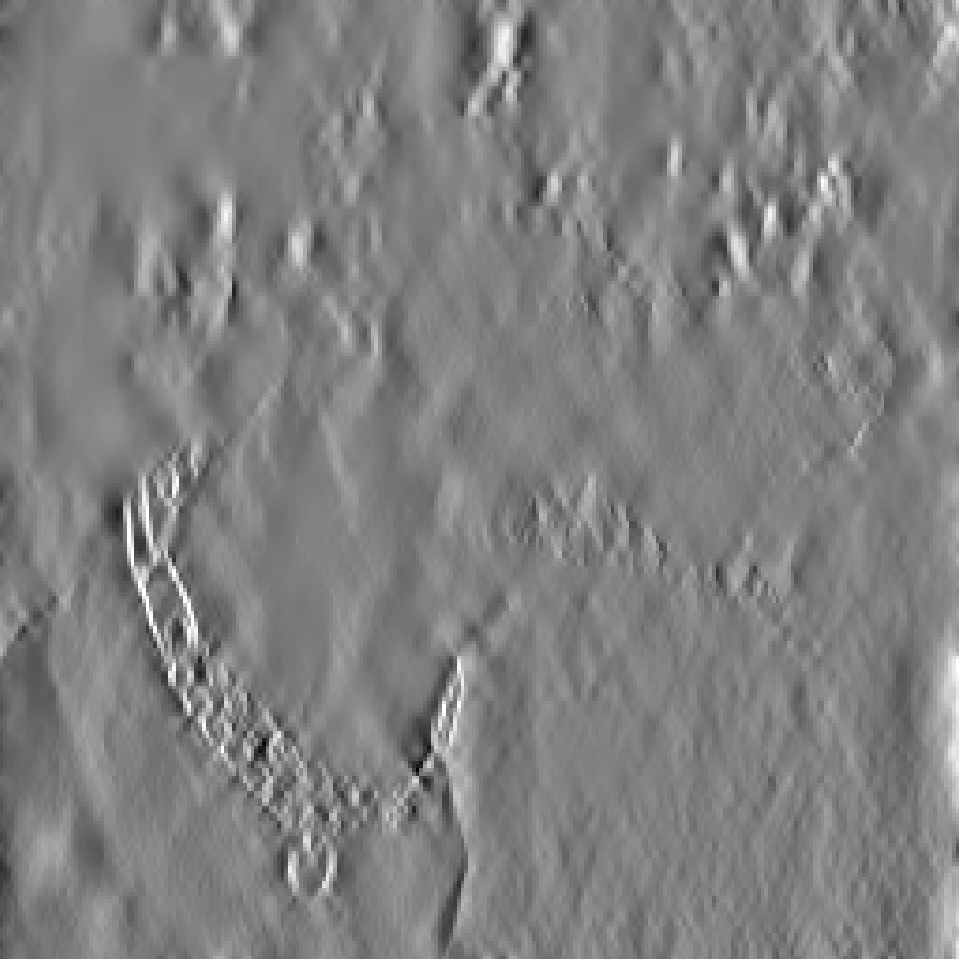hg4 <- matrix(0, nrow=256, ncol=256) hg4[17:32, 1:16] <- wt.img.4$y[17:32, 1:16]
hg4[33:64, 1:32] <- wt.img.4$y[33:64, 1:32] hg4[65:128, 1:64] <- wt.img.4$y[65:128, 1:64]
hg4[129:256, 1:128] <- wt.img.4$y[129:256, 1:128] hg4_signal <- midwt(hg4, daubcqf(8)$h.0, L=4)
img.plot(hg4_signal$x)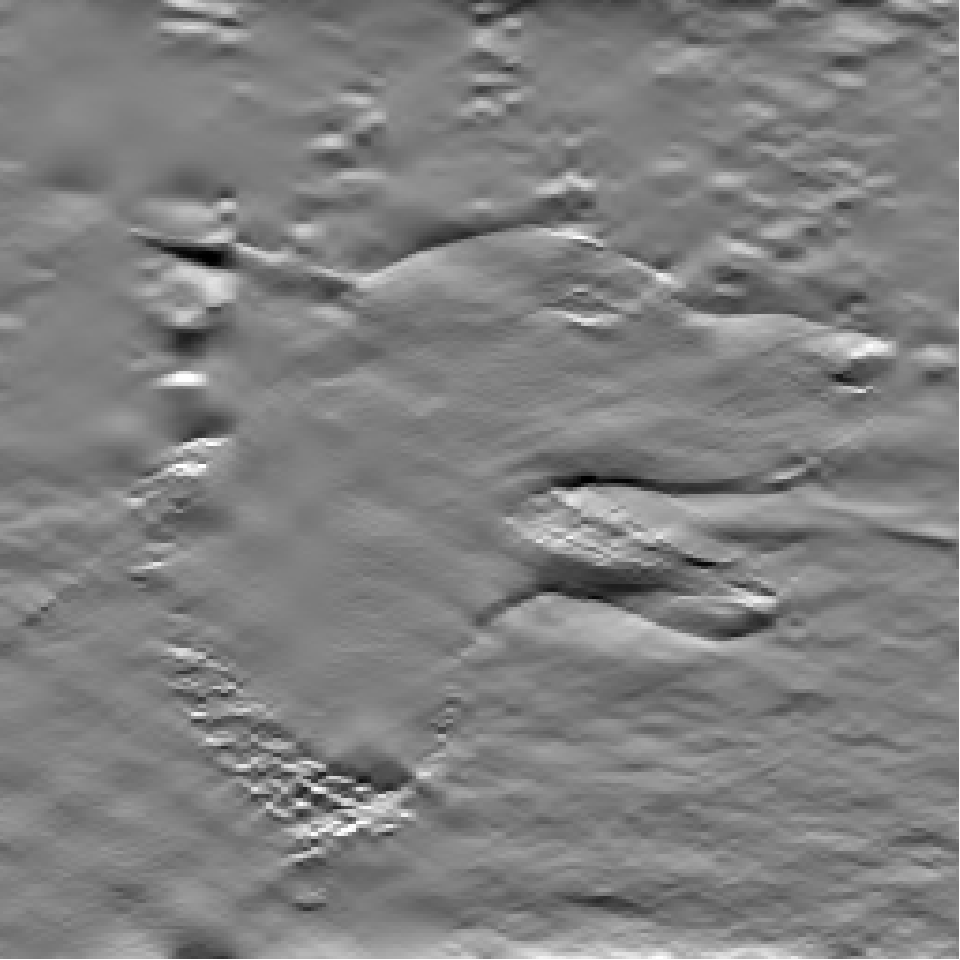hh4 <- matrix(0, nrow=256, ncol=256) hh4[17:32, 17:32] <- wt.img.4$y[17:32, 17:32]
hh4[33:64, 33:64] <- wt.img.4$y[33:64, 33:64] hh4[65:128, 65:128] <- wt.img.4$y[65:128, 65:128]
hh4[129:256, 129:256] <- wt.img.4$y[129:256, 129:256] hh4_signal <- midwt(hh4, daubcqf(8)$h.0, L=4)
img.plot(hh4_signal$x)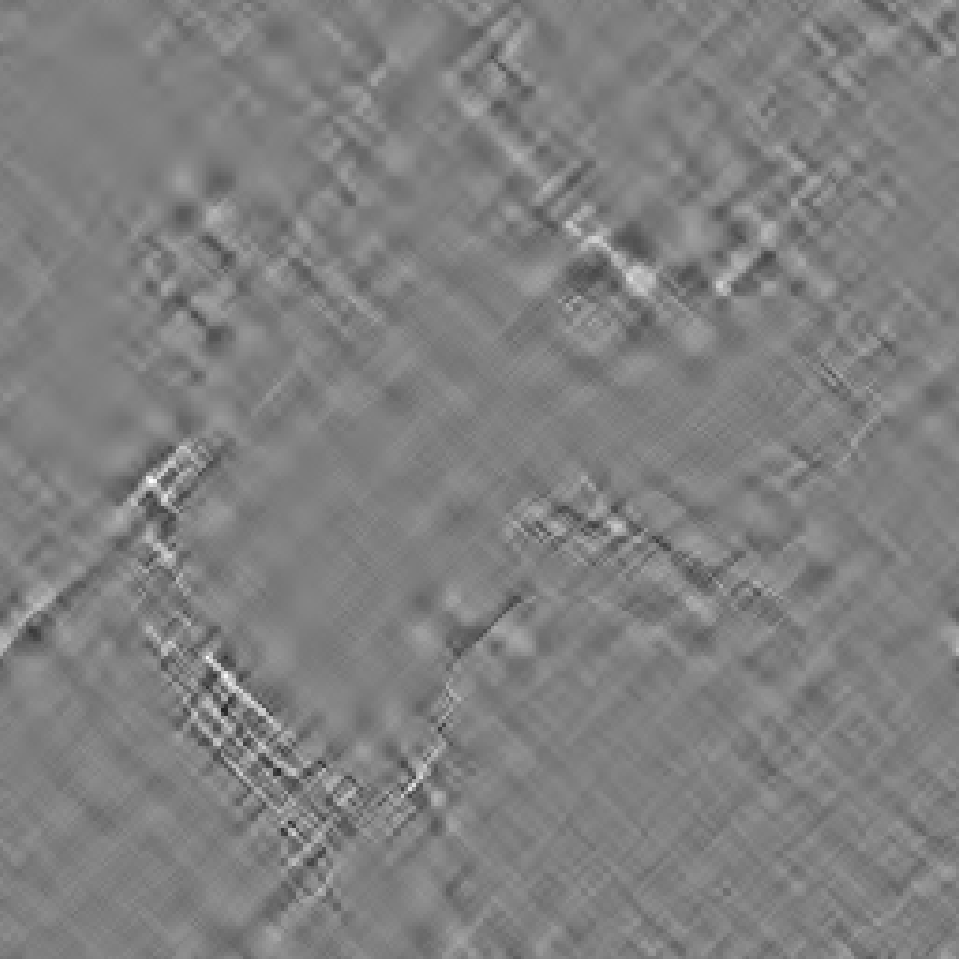detail4 <- gh4 + hg4 + hh4 detail4_signal <- midwt(detail4, daubcqf(8)$h.0, L=4)
img.plot(detail4_signal$x)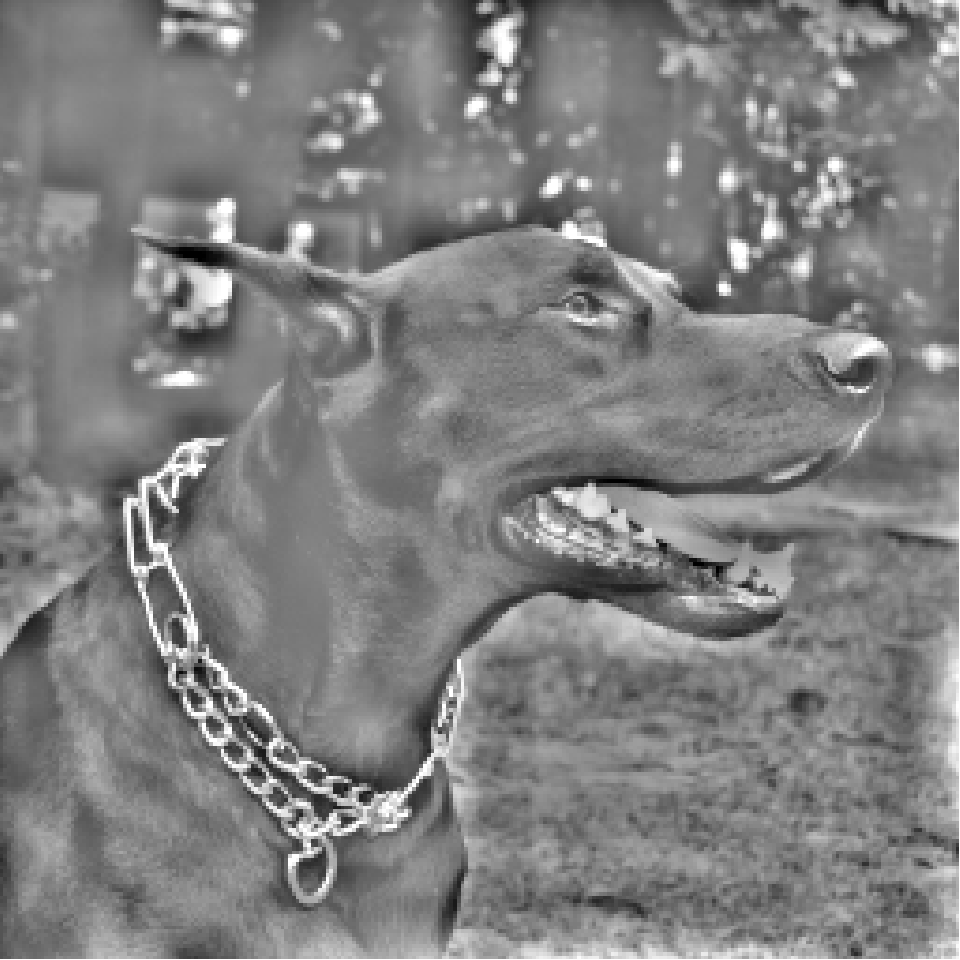pctl_99 <- quantile(abs(wt.img.4$y), probs=0.99)
top_01 <- matrix(0, nrow=256, ncol=256)
top_01[abs(wt.img.4$y)>pctl_99] <- wt.img.4$y[abs(wt.img.4$y)>pctl_99] top_01_image <- midwt(top_01, daubcqf(8)$h.0, L=4)
img.plot(top_01_image$x)pctl_95 <- quantile(abs(wt.img.4$y), probs=0.95)
top_05 <- matrix(0, nrow=256, ncol=256)
top_05[abs(wt.img.4$y)>pctl_95] <- wt.img.4$y[abs(wt.img.4$y)>pctl_95] top_05_image <- midwt(top_05, daubcqf(8)$h.0, L=4)
img.plot(top_05_image$x)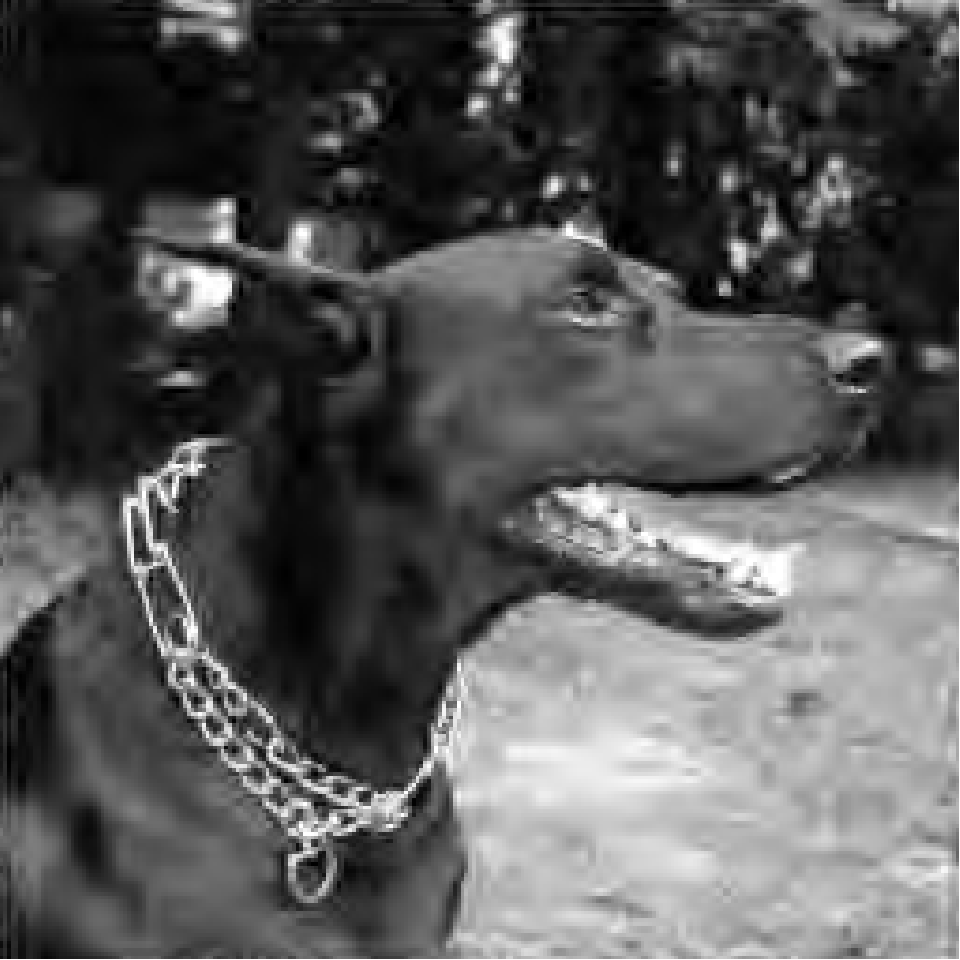pctl_90 <- quantile(abs(wt.img.4$y), probs=0.90)
top_10 <- matrix(0, nrow=256, ncol=256)
top_10[abs(wt.img.4$y)>pctl_90] <- wt.img.4$y[abs(wt.img.4$y)>pctl_90] top_10_image <- midwt(top_10, daubcqf(8)$h.0, L=4)
img.plot(top_10_image\$x)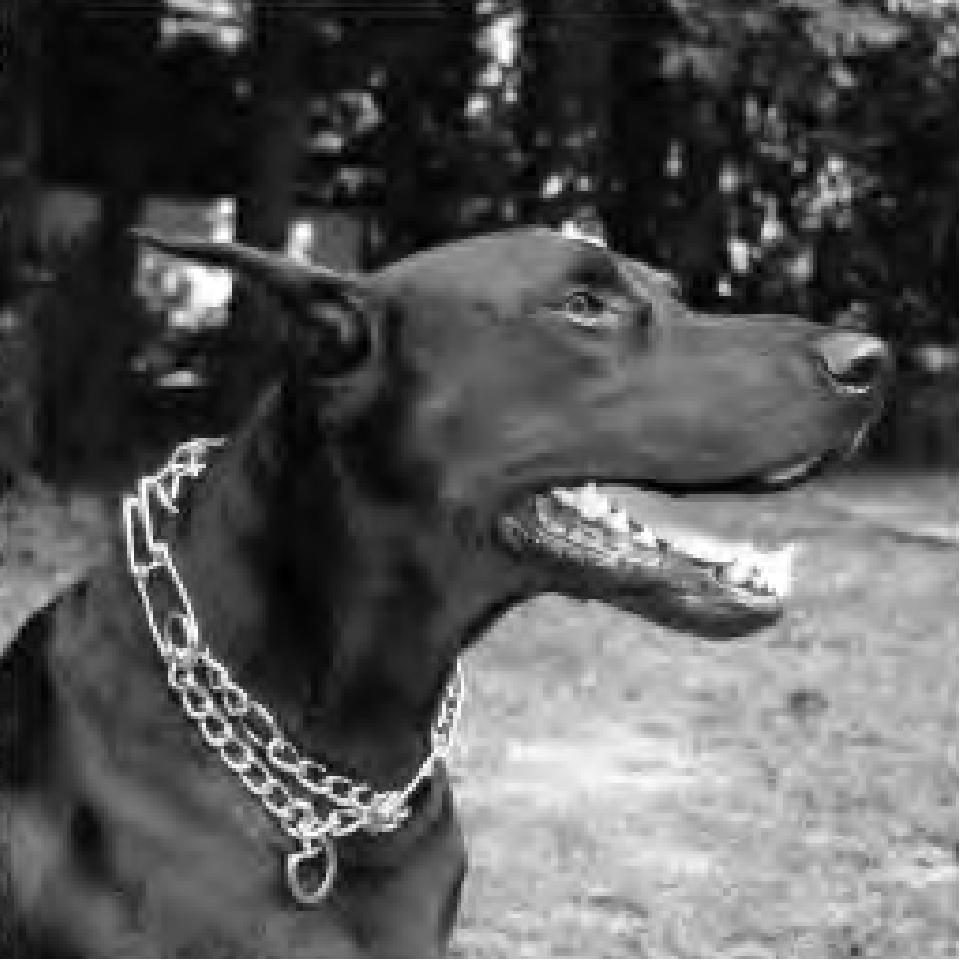Although there is some arbitrariness here, you can assign the outer(h,g) wavelets along the top.

Layout of horizontal wavelets the outer(g,h) wavelets along the side

Wavelet layout

and the outer(h,h) wavelets along the diagonal.

Wavelet layout

If you examine the contribution of each wavelet frequency to the image, you will see a distinct pattern.

W1

This W1. The gray areas represent the average color and regions of the image where no high frequency events occur. You can see high frequency events around the dog’s collar and the dog’s face.

W2

This is W2. It looks a lot like W1 but is a bit broader.

W3

This is W3. You see a bit more activity in the background, representings shifts in the color of the background image.

W4

This is W4. It looks like a blurred image with undulating colors rather than sharp shifts.

W5

This is W5. It is an amorphous blob representing graduals shifts in the average color.

W6

This is W6. It is just like W5 at an even broader scale.

If you look at the original image again, you will see regions (mostly in the background surrounding the dog or in large patches of the dog’s fur) where the color is mostly the same. These are captured by the low frequency wavelets. The sudden transitions in color, especially around the dog’s collar, are captured in high frequency wavelets. You can look at the cumulative effect of the wavelets as well.

W2

Here is W1 again.

W1 and W2

Here is W1 plus W2.

W1 through W3

Here is W1 through W3. Notice that the edges around the collar and the dog’s face are well defined here. Also notice the large patches of gray. Gray is zero and it represents the average color between white and black. The areas in gray have no sharp edges and are filled in primarily with the lower frequency wavelets.

W1 through W4

Here is W1 through W4. At this level, some of the broad color regions are starting to become apparent, though the image is still largely washed out.

W1 through W6

Here is W1 through W5. The image is very similar to the original, but there are some patches that V5 would be needed to fill in.

You can also look at the reconstructed image using V1 through V6.

V1

Here is V1. It is the full image with just a few very sharp edges removed by W1.

V2

Here is V2. With removal of another set of edges by W2, the picture starts to take on a slightly blurry feel.

V3

Here is V3. It has an even greater blur.

V4

Here is V4. The dog is blurred almost beyond recognition.

V5

Here is V5. There is nothing recognizable here except general patterns of dark and light through broad regions of the image.

V6

Finally, here is V6. It is pretty much the same as V6, but at an even broader scale.

You can also reconstruct the three different sets of wavelet coefficients separately.

W1 through W3 horizontal

Here is an image reconstructed with the W1 through W3 of horizontally oriented wavelets only.

W1 though W3 vertical

Here is the reconstruction using W1 through W3 of the vertically oriented wavelets only.

W1 through W3 diagonal

Here is the reconstruction using W1 through W3 of the diagonally oriented wavelets only.

There seems to be more detail in the horizontally oriented wavelets. That’s because the mouth, the eye and the ear are mostly horizontal. The edge of the dog’s body, however, is vertical and is prominent only in the second image. The collar is a mix of horizontal, vertical, and diagonal elements, so it is apparent in each of the three images.

There’s a lot more to wavelets, but this should help get you started. Now that you know how to compute the wavelet decomposition in one and two dimensions, you are ready to start applying them to real situations.

There are several important applications of wavelets. First, the wavelet coefficients themselves tell you information about the nature of the signal or image. Are there high frequency events or low frequency events (or both) and are those restricted to a particular part of the signal.

Second, you can use wavelets to get a “de-noised” signal. Those small coefficients that are (presumably) just noise. If you reconstruct the signal or image without those small coefficients (by rounding them down to zero), you will often get a “cleaner” estimate of the signal. Wavelets do an especially good job of de-noising when the signal is not smooth. Smooth means a discontinuous first derivative, but in most applications it represents an abrupt turn in the signal. Wavelets even can work well with discontinuous signals.

In contrast, the Fourier decomposition has lots of problems with non-smooth, but especially discontinuous functions. The problems go under the general name of the Gibbs phenomenon.

Third, you can zero out the small coefficients and store only the large coefficients. This allows you to reconstruct a good approximation of the image while saving substantially on storage. This is really only used for images, because one dimensional signals, even very long ones just don’t take up a lot of room. The jpg 2000 standard used wavelets for compression.

Fourth, wavelets can help you detect edges in an image. An edge is an abrupt transition from light to dark, or dark to light. The high frequency wavelets help you detect where the edges are in an image.

Wavelets have a couple important limitations. The first is a minor issue, but the wavelets need to be adapted when the number of points in your signal is not a simple power of 2 or when the two dimensions of length and width of your image are not both simple powers of 2. A more important problem is that wavelets rely on equally spaced sample of the signal or image. Subsampling or interpolation can sometimes help here.

save(list=ls(), file="wavelets.RData")

You can find an earlier version of this page on my old website.